# NCERT Exemplar Solutions for Class 11 Maths Chapter 14 Mathematical Reasoning

NCERT Exemplar Solutions for Class 11 Chapter 14 Mathematical Reasoning covers all the questions provided in the NCERT Exemplar textbook. These NCERT Exemplar Solutions, prepared by experts at BYJU’S, are a comprehensive study material for the students preparing for the Class 11 examination. These solutions are available for easy access and download of the students. NCERT Exemplar books have been prescribed for years as a complete source of information to CBSE students, to develop their analytical skills. They have proven to be essential for learning the syllabus and developing the confidence that is required to face their exams. The NCERT Exemplar Solutions explain the steps with precision, without missing out on essential aspects of solving a question.

The 14th Chapter Mathematical Reasoning of NCERT Exemplar Solutions for Class 11 Maths explains mathematical statements. The main aim of these solutions is to provide a fundamental aspect of Maths, which in turn, helps the students to understand every concept clearly. Some of the essential topics of this chapter are listed below:

• Definition of statements
• Simple statements
• Compound statements
• Basic logical connectives
• Definition and meaning of conjunctions
• Definition and meaning of disjunction
• Negation
• Negation of compound statements
• Negation of conjunction
• Negation of disjunction
• Negation of a negation
• The conditional statement
• Contrapositive of a conditional statement
• Converse of a conditional statement
• The biconditional statement
• Quantifiers
• Validity of statements
• Validity of the statement with “If and only If”

## Download the PDF of NCERT Exemplar Solutions For Class 11 Maths Chapter 14 Mathematical Reasoning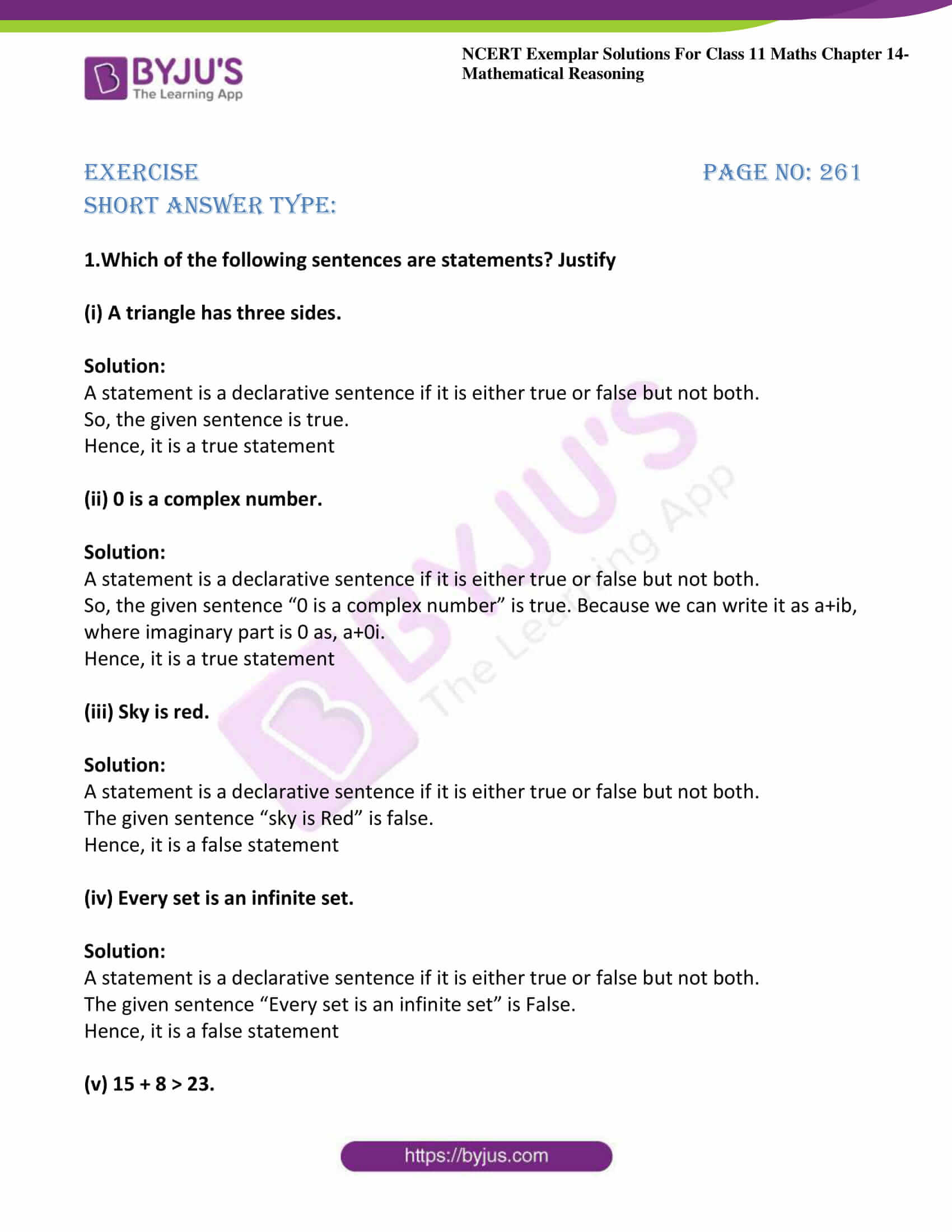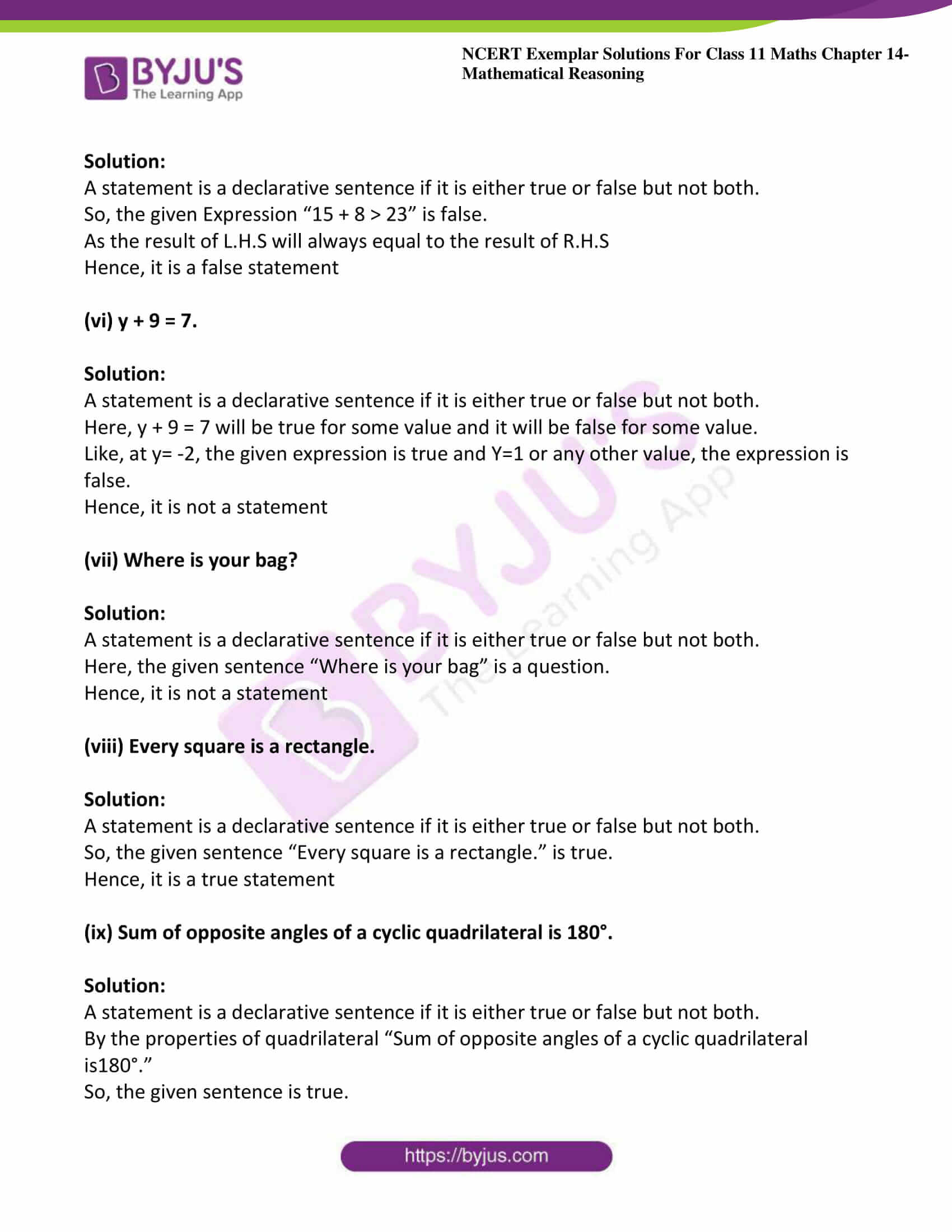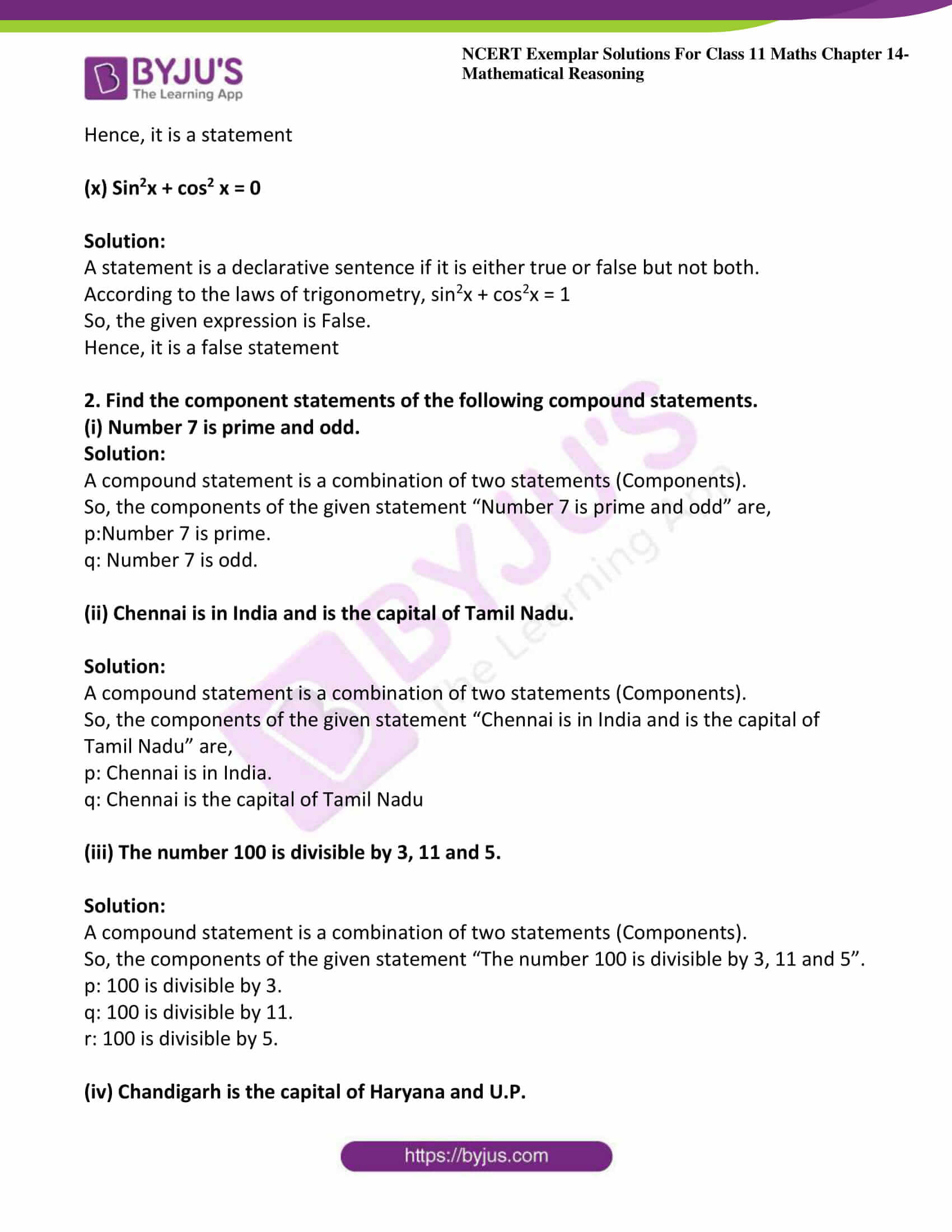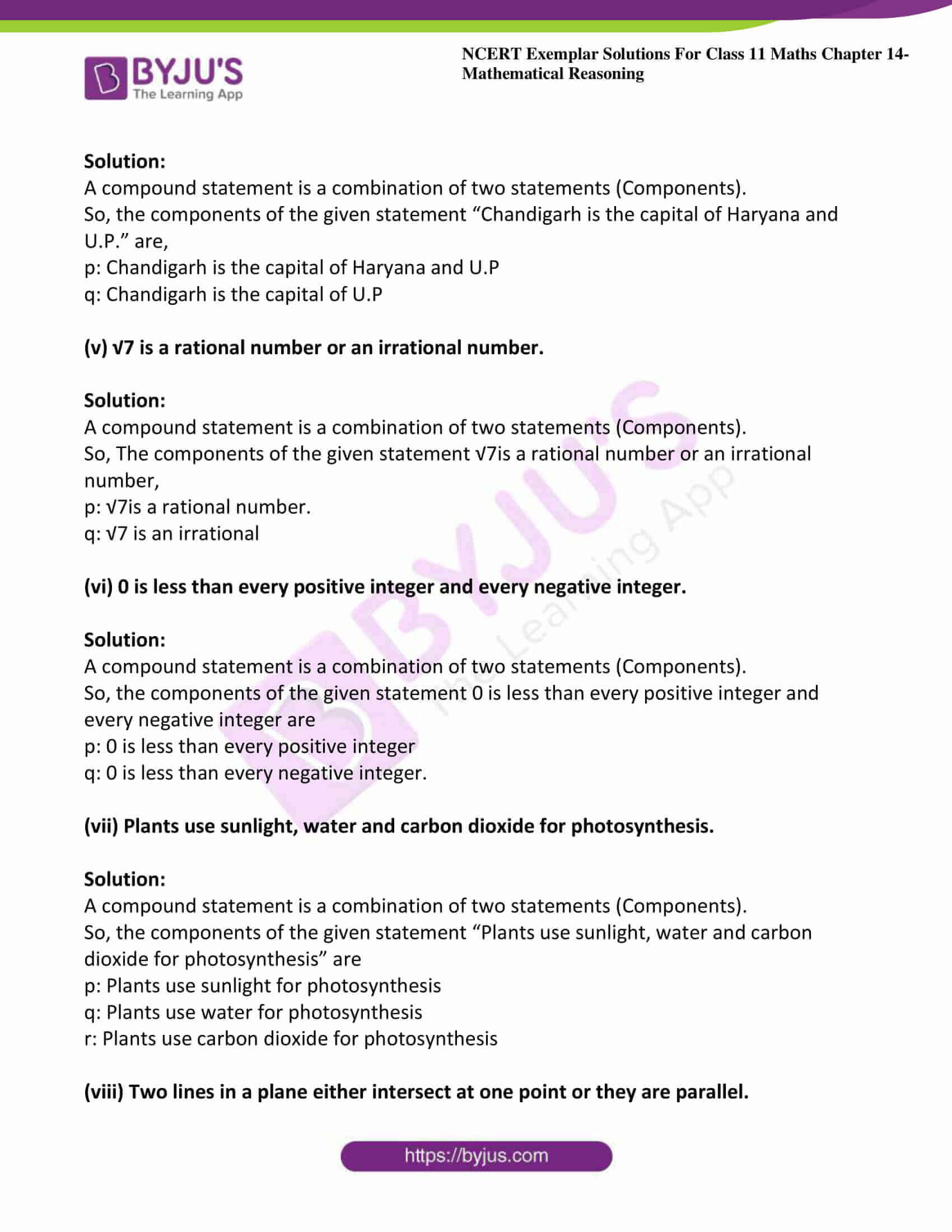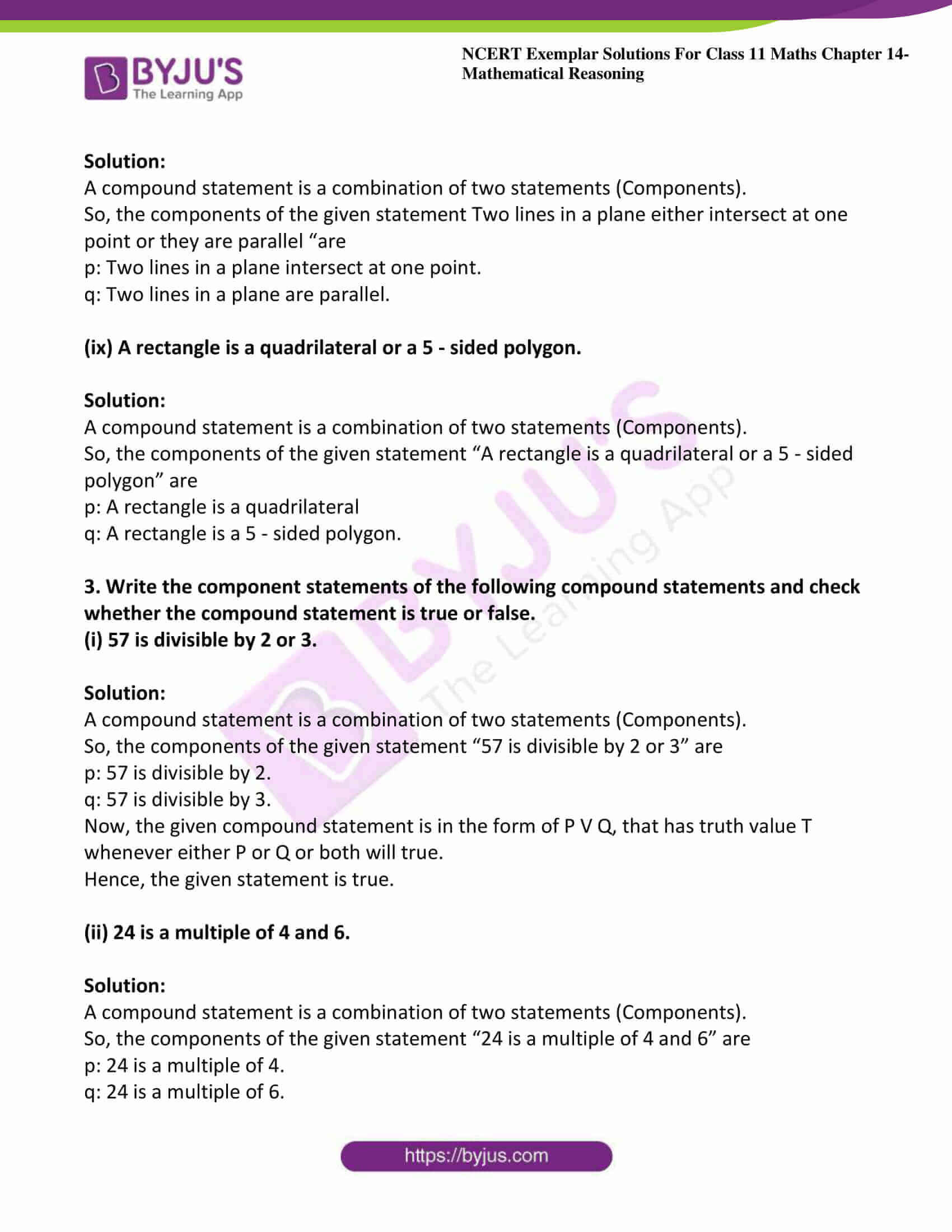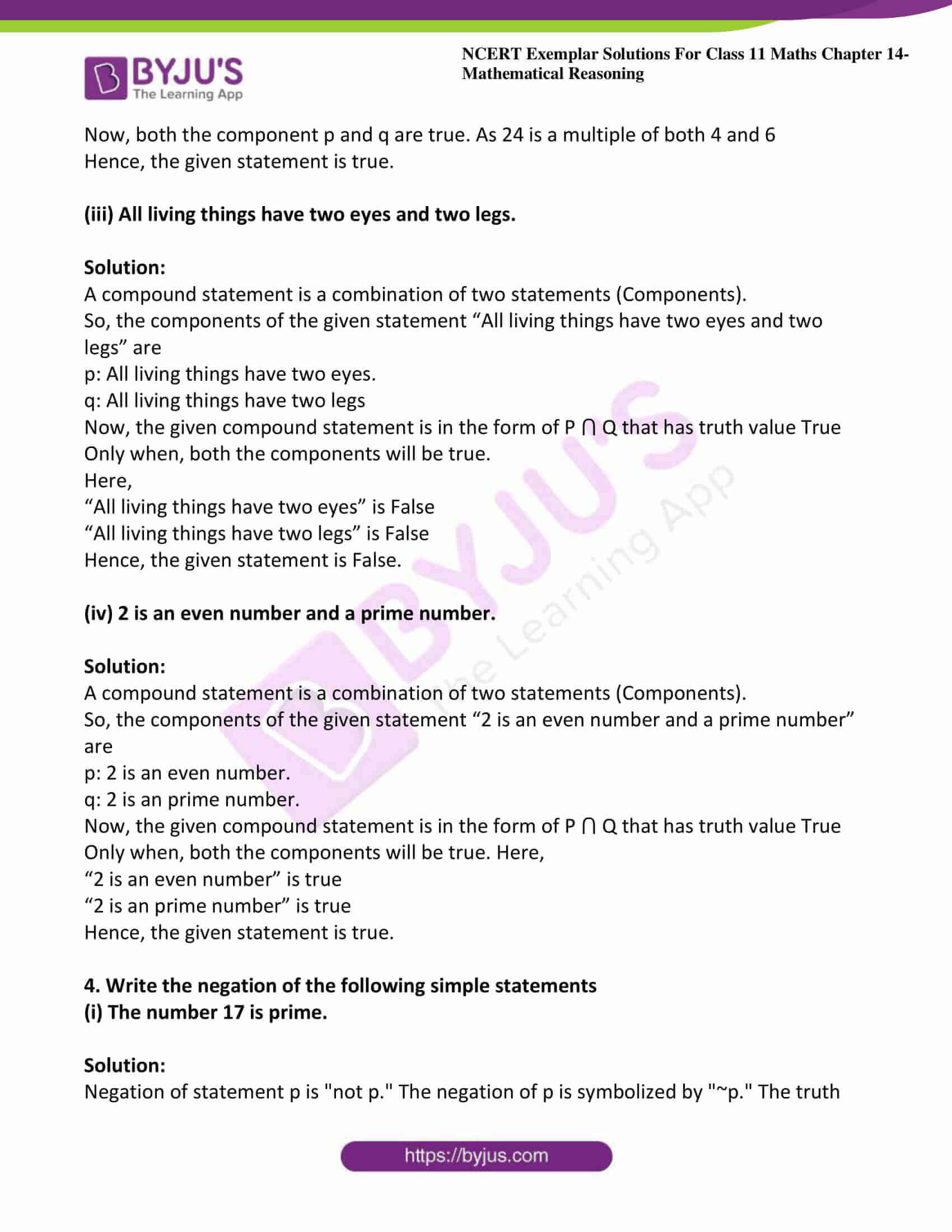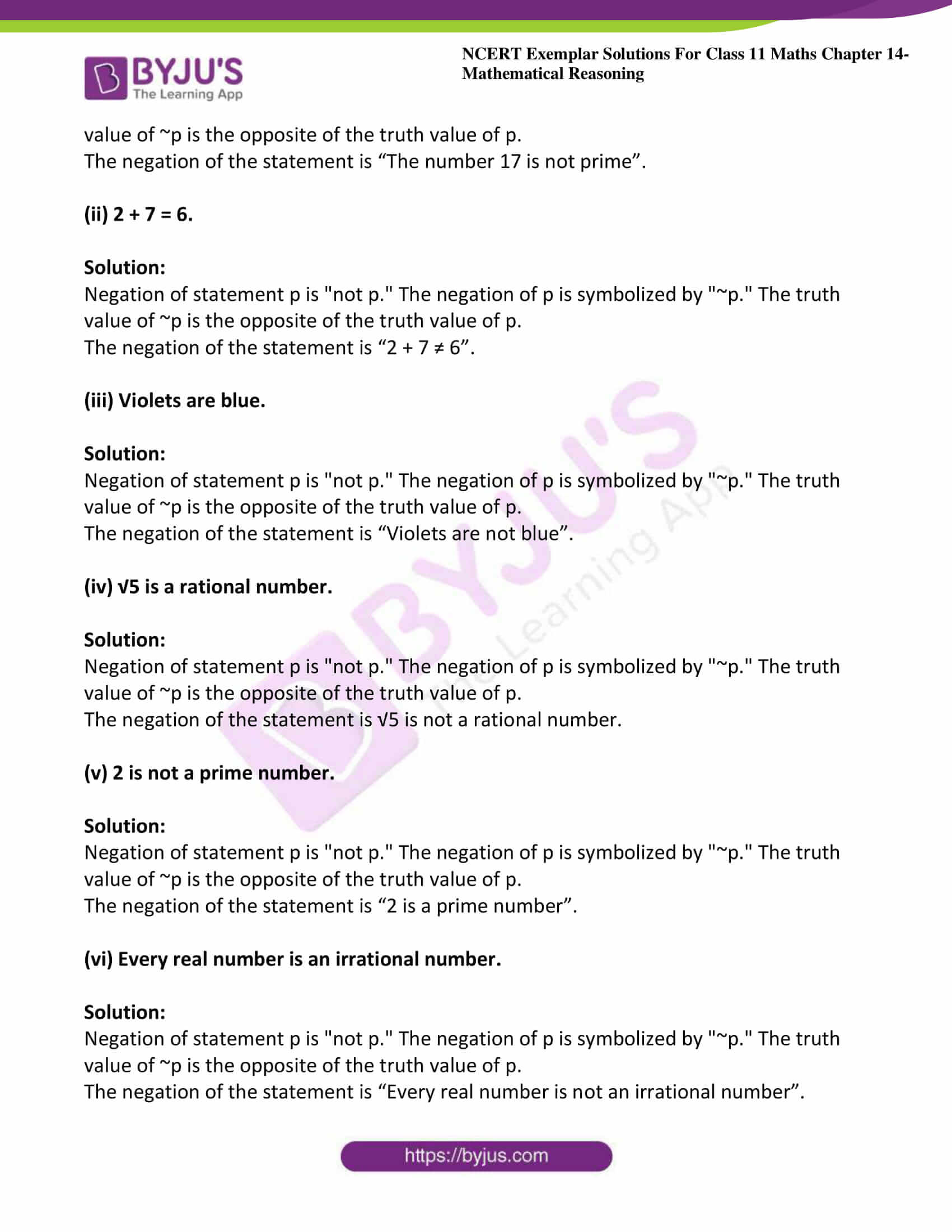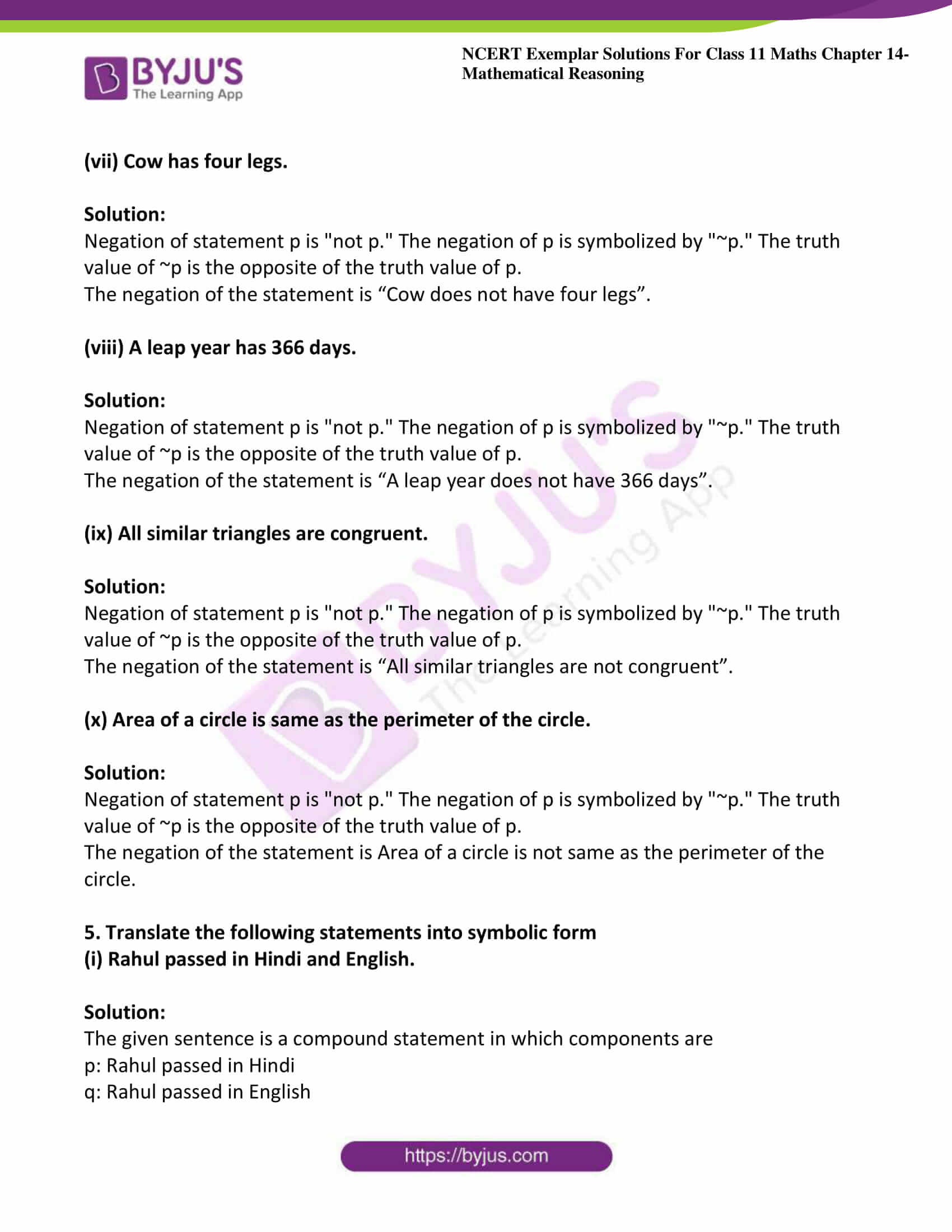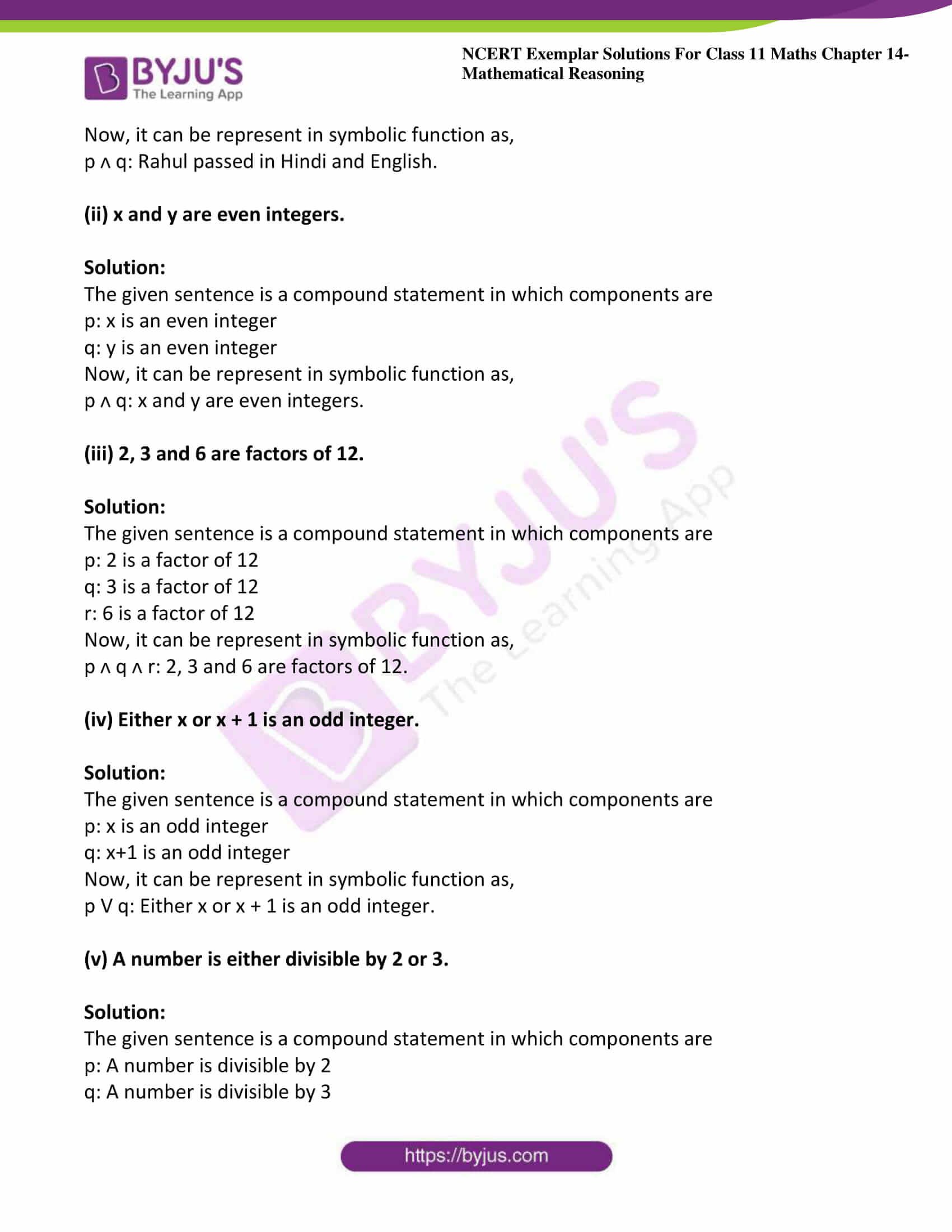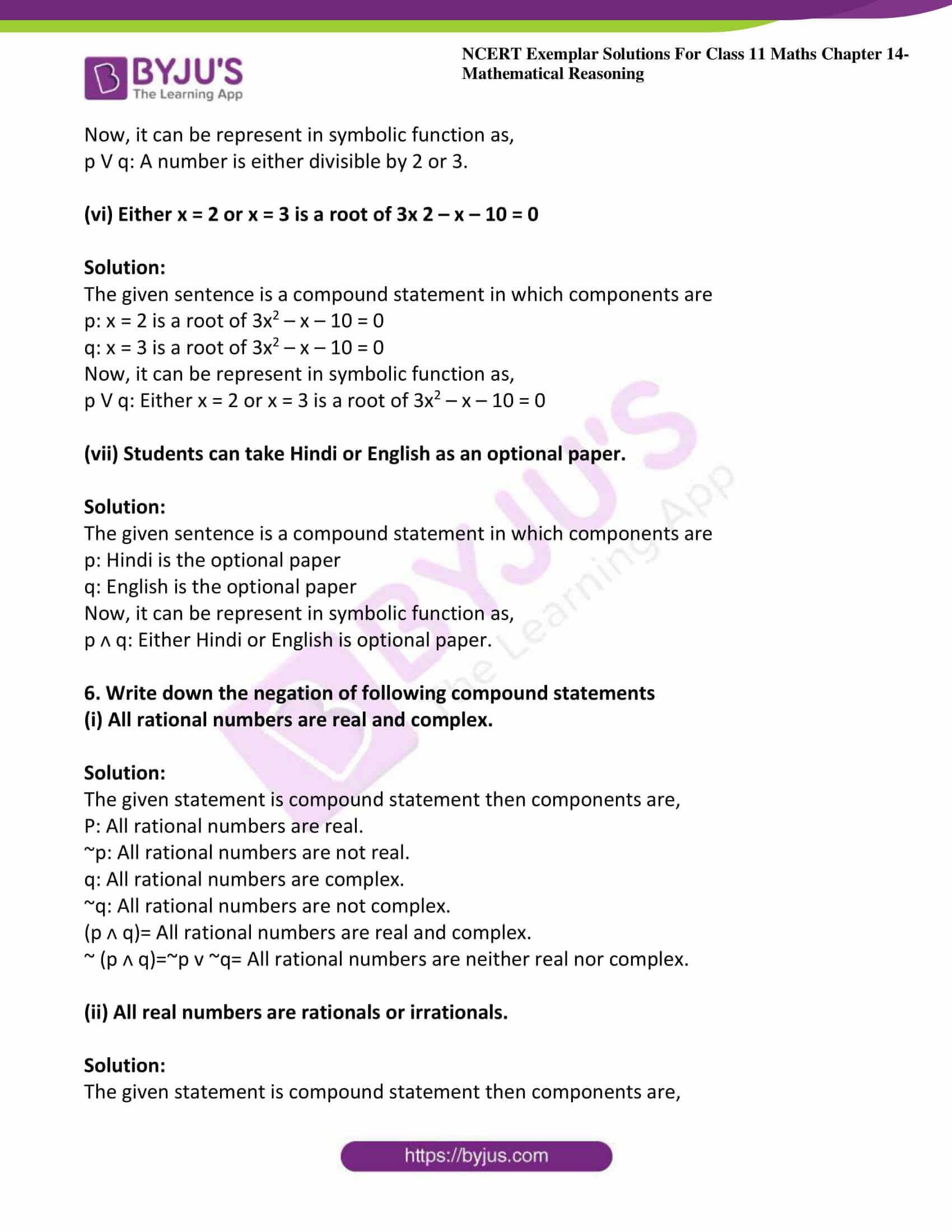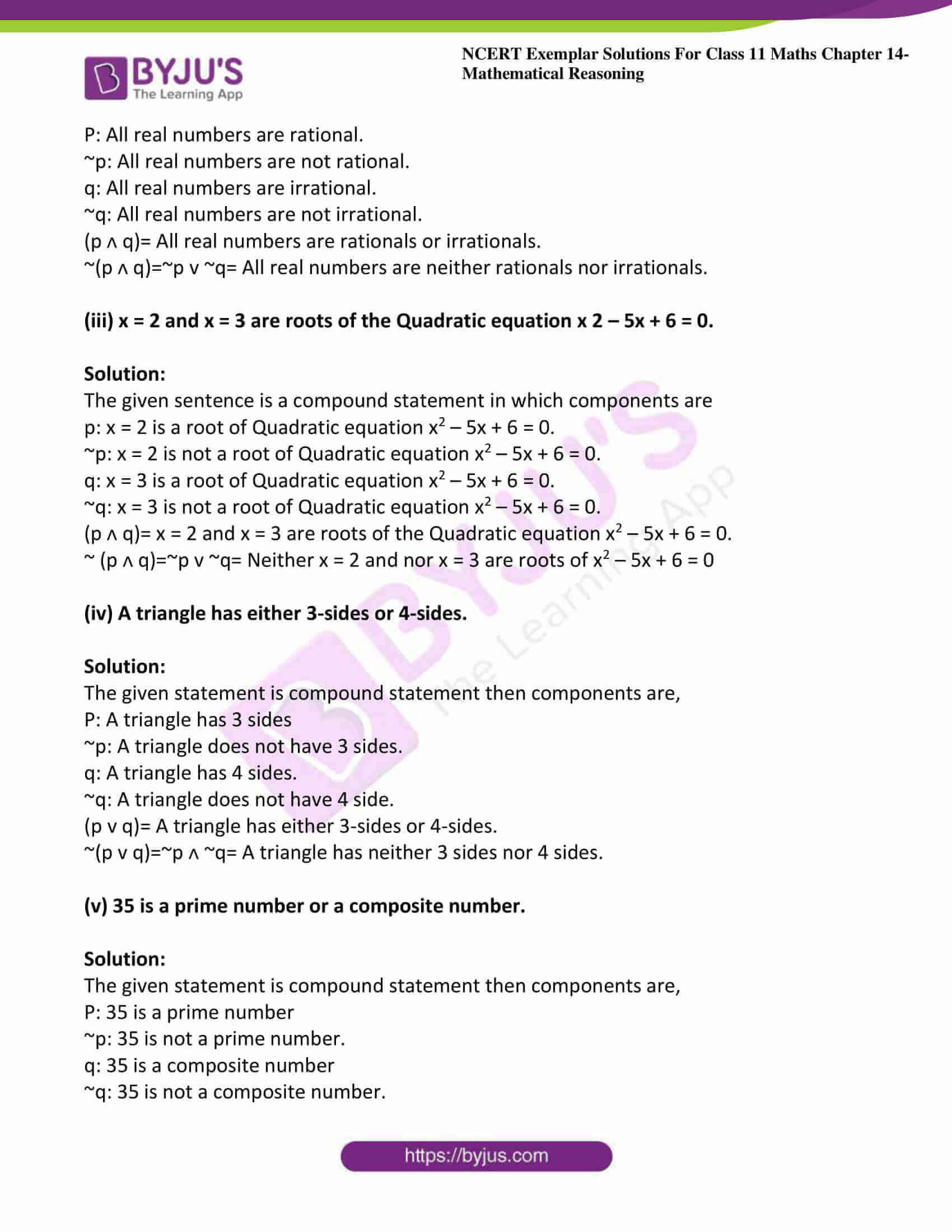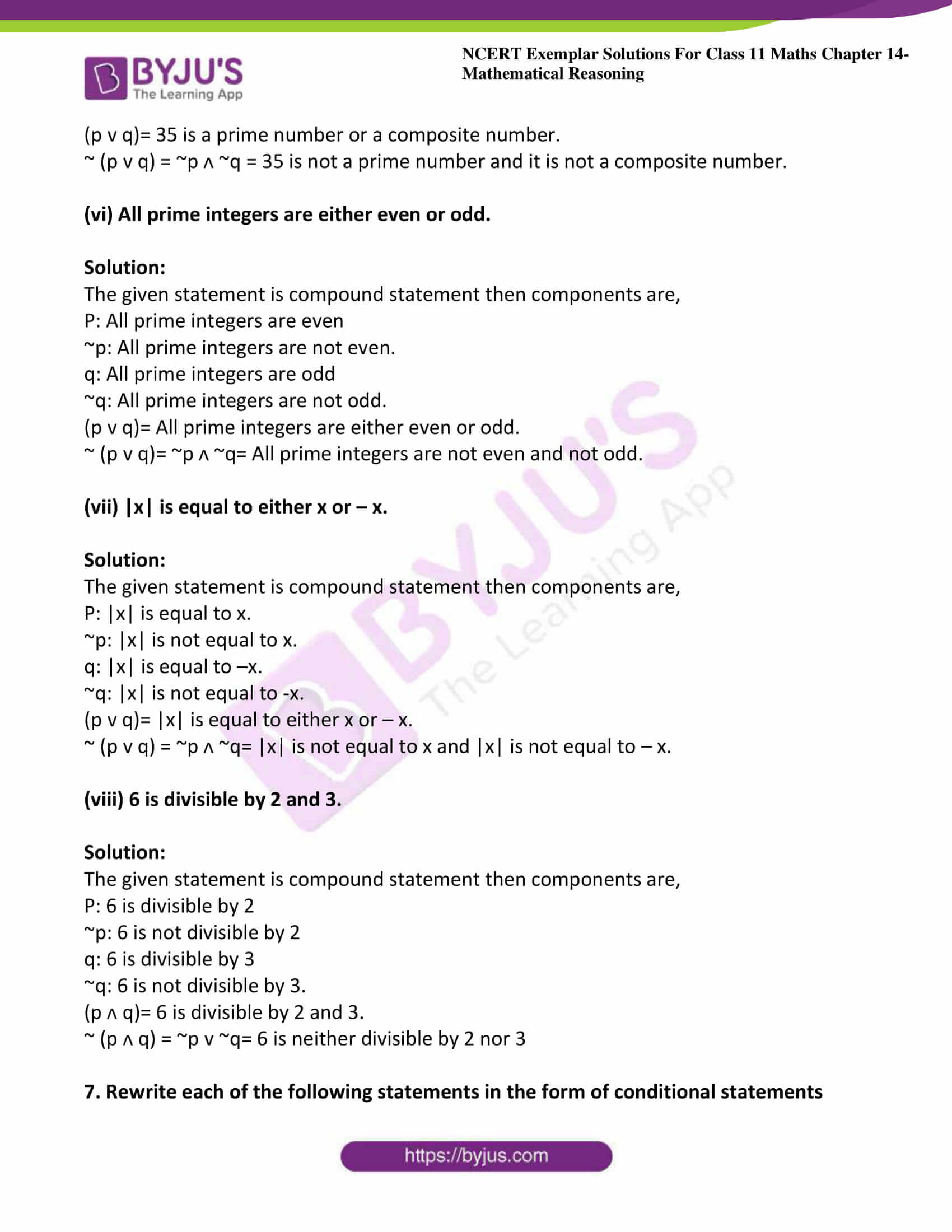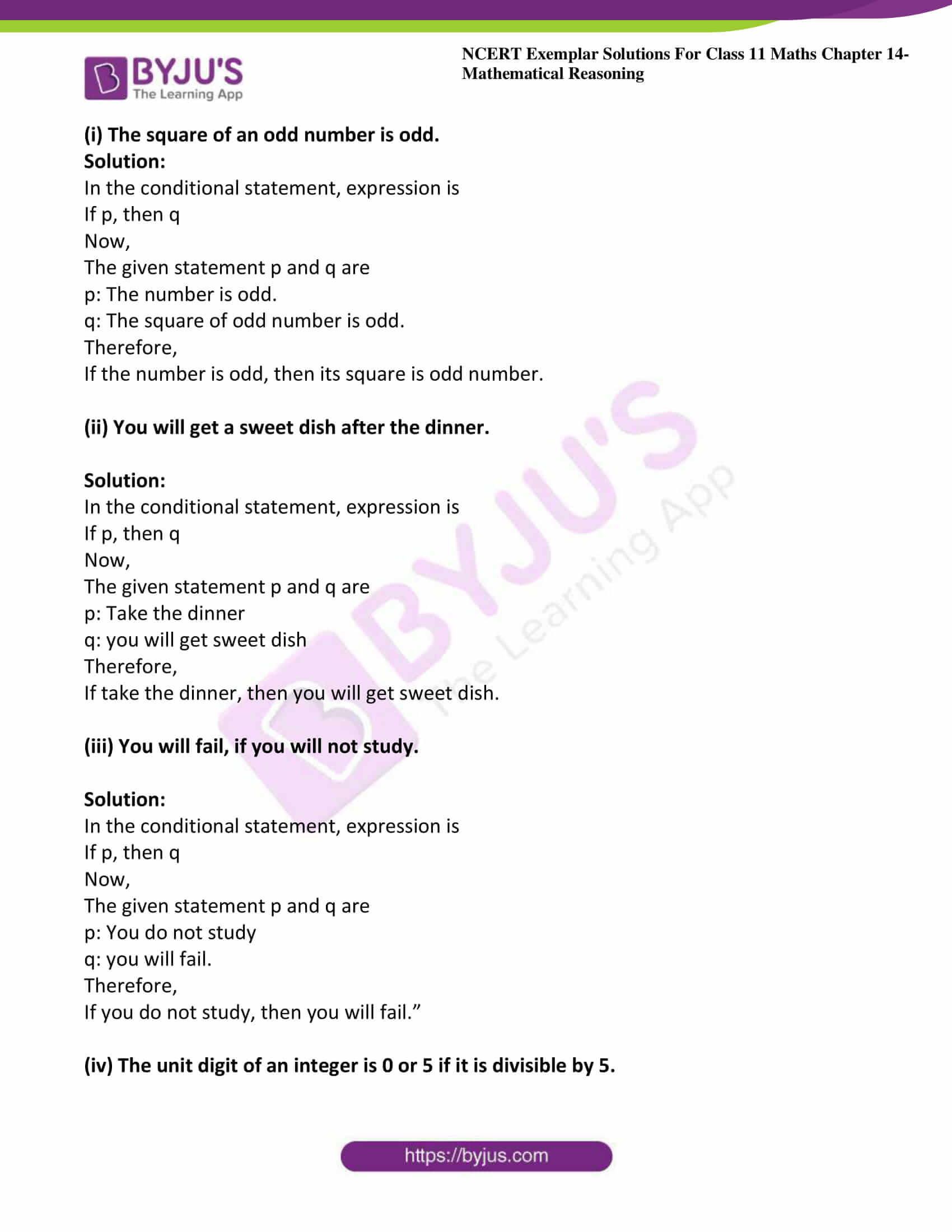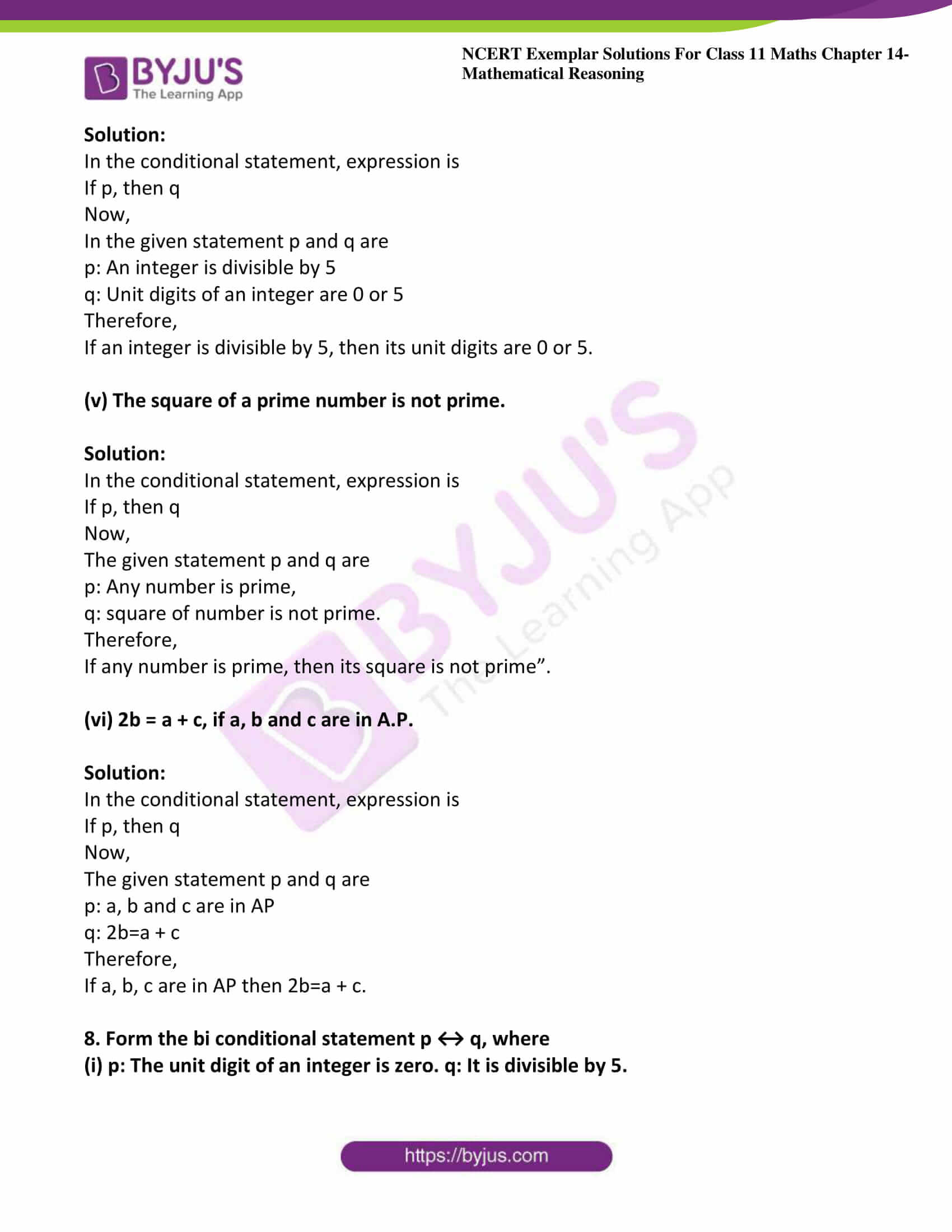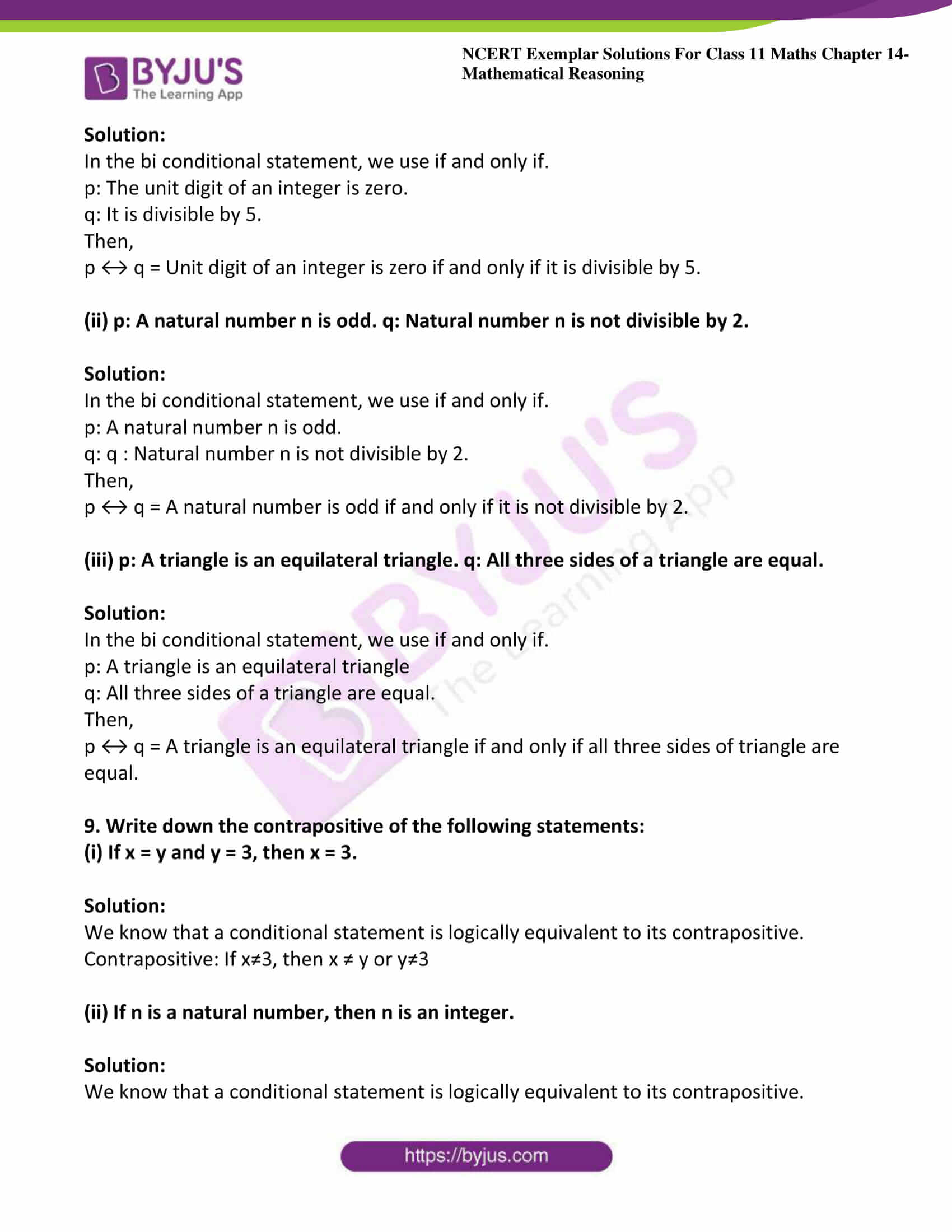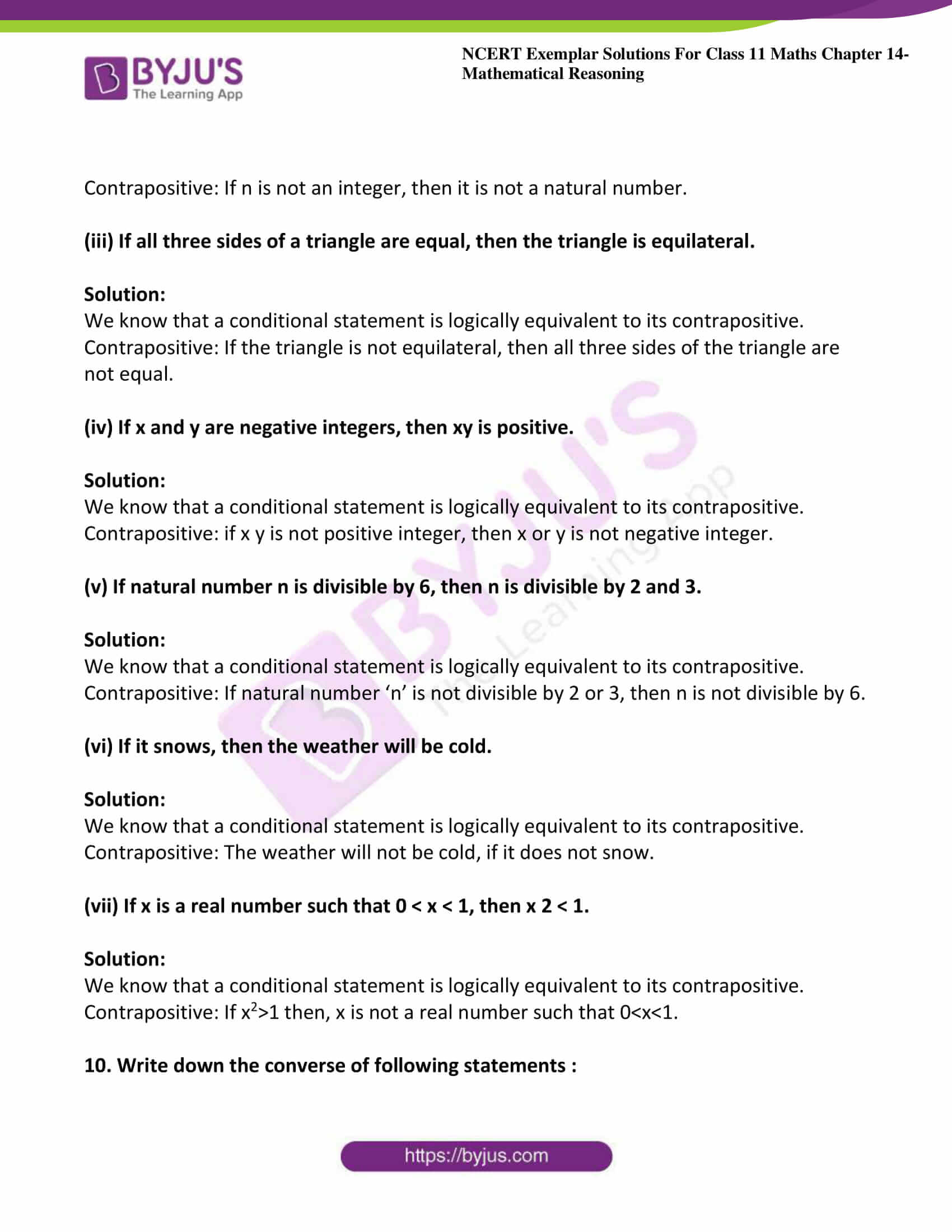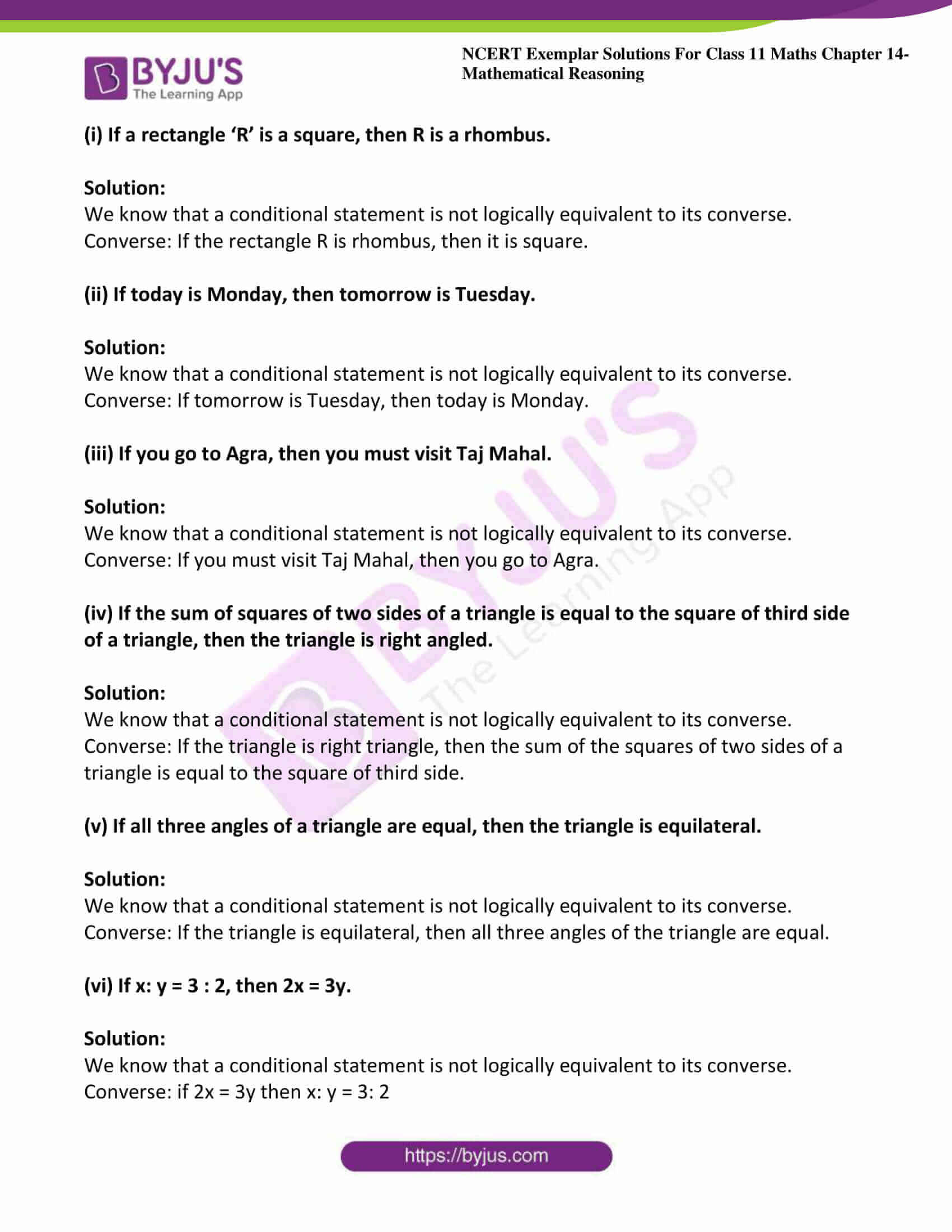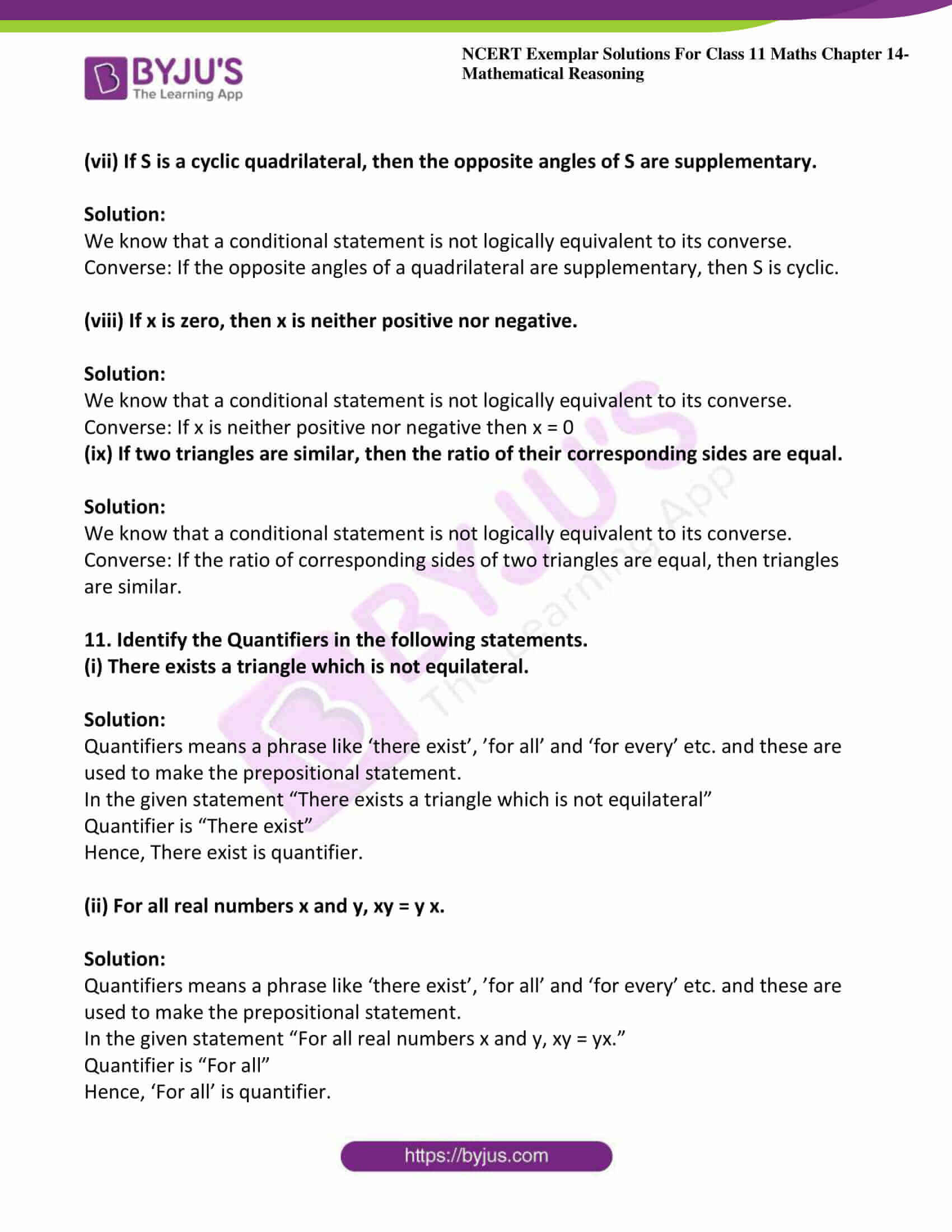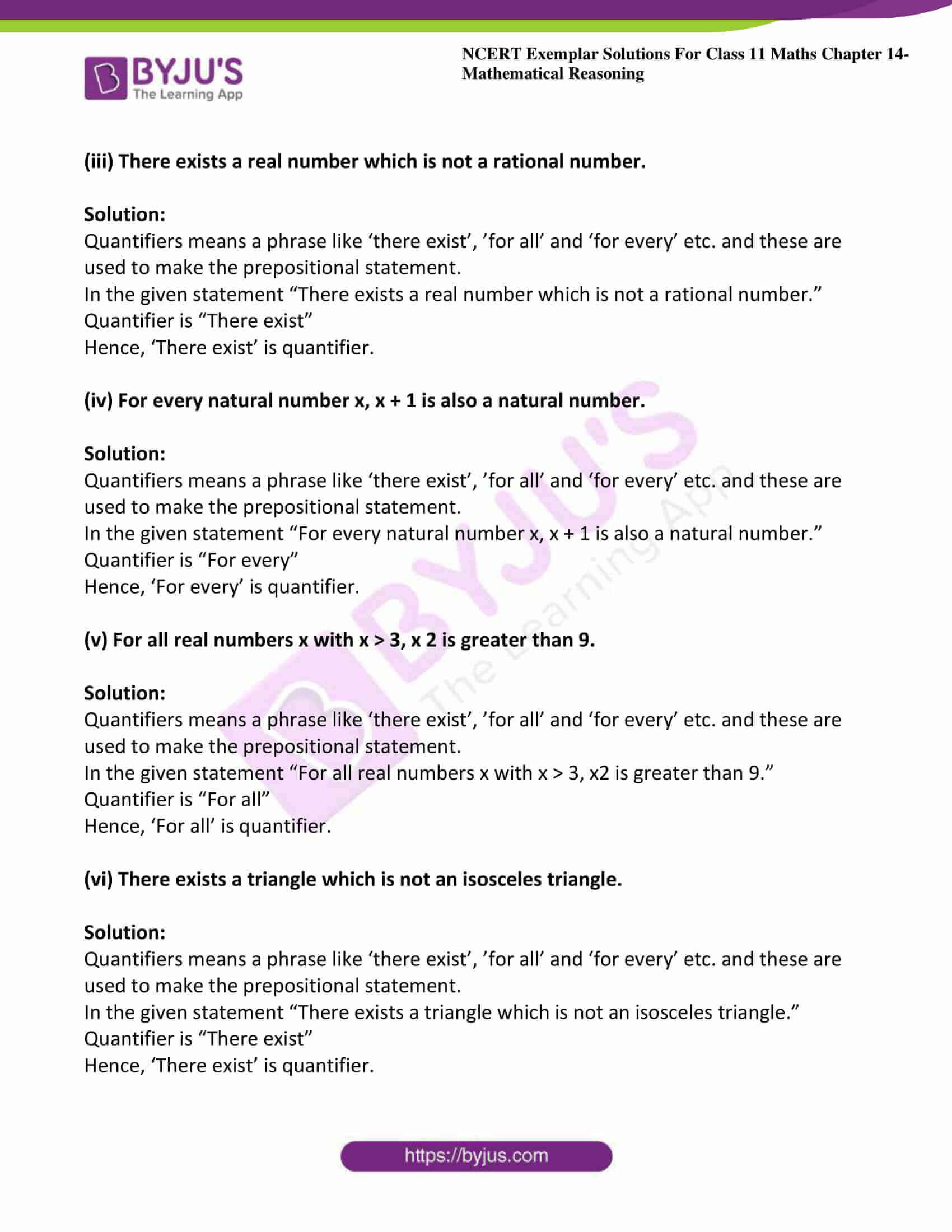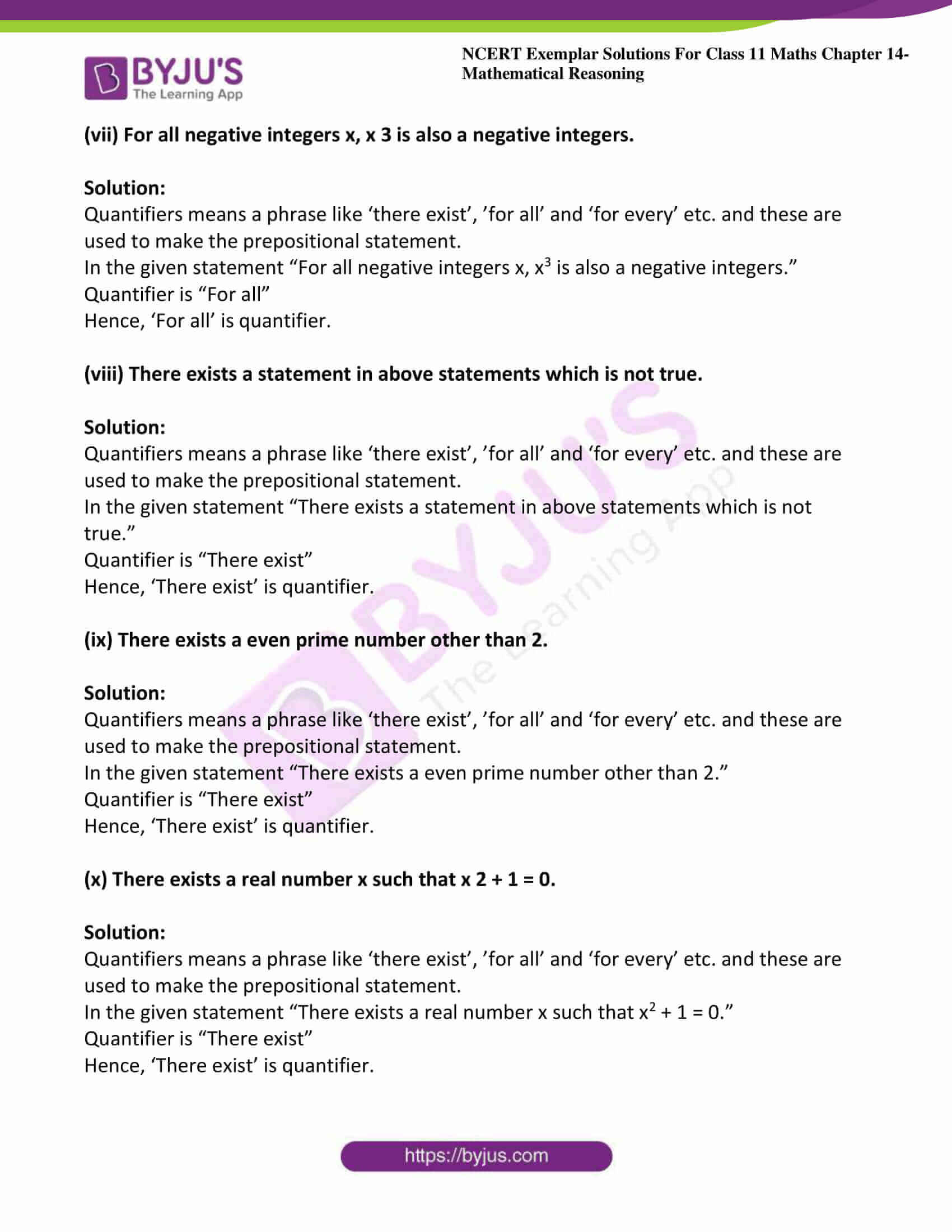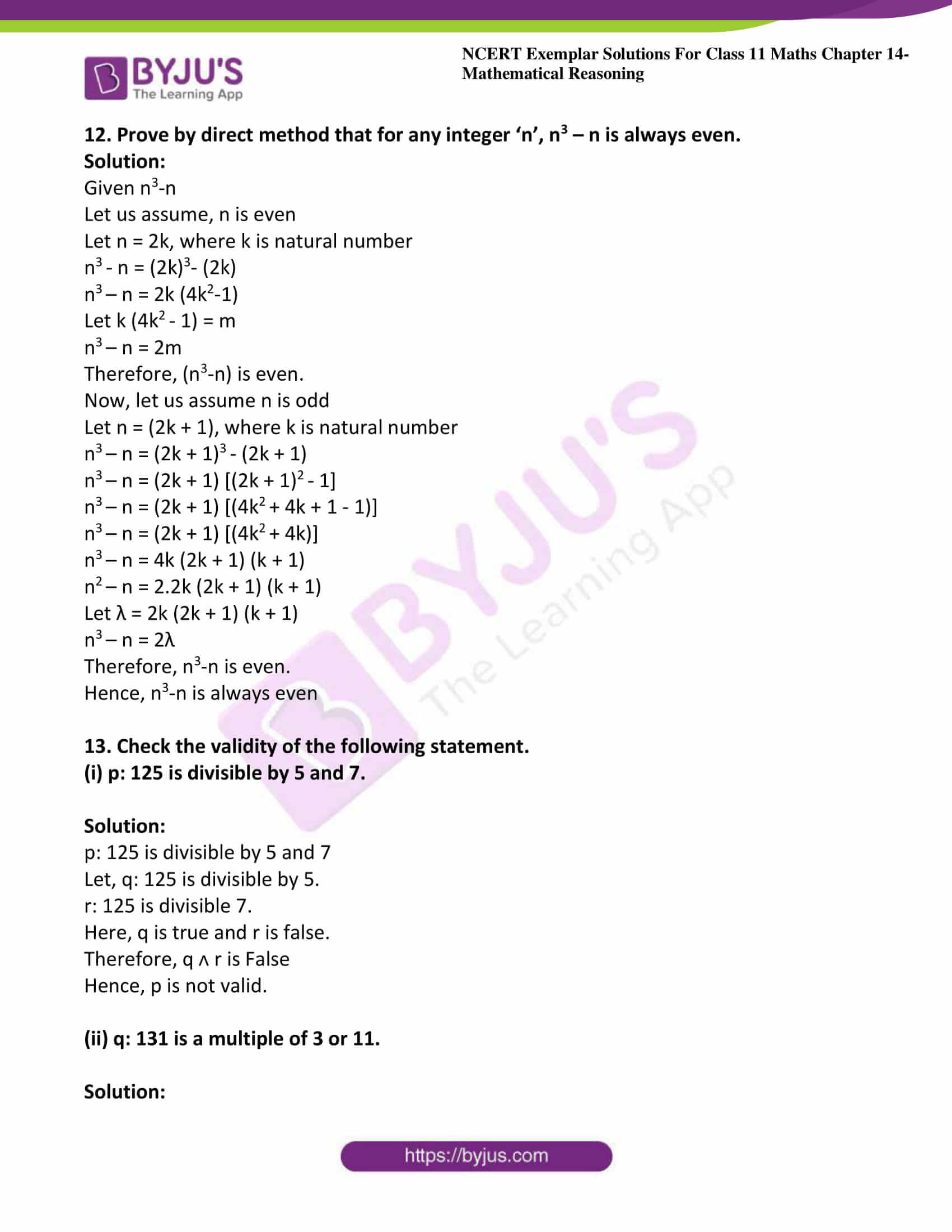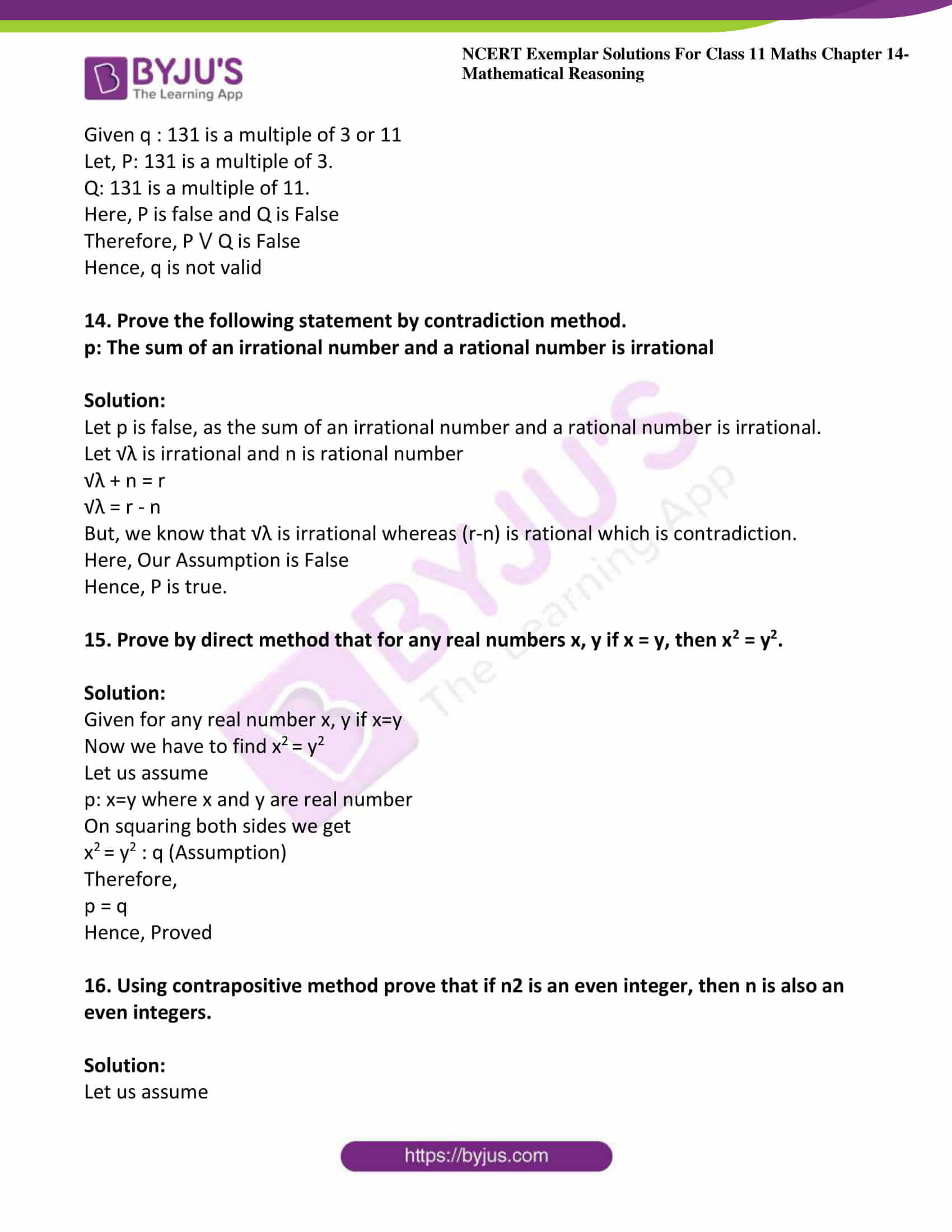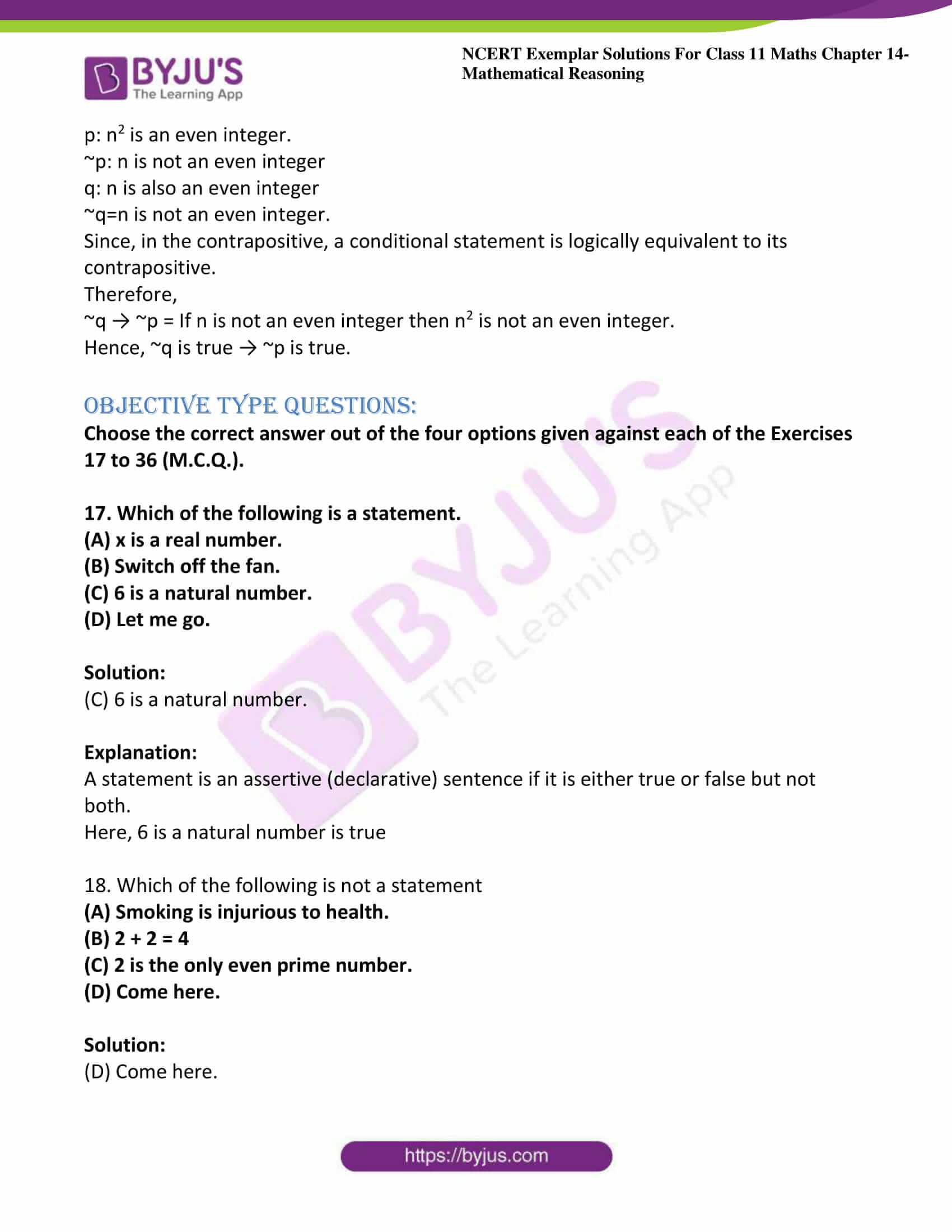### Access Answers to NCERT Exemplar Class 11 Maths Chapter 14 – Mathematical Reasoning

Exercise Page No: 261

1.Which of the following sentences are statements? Justify

(i) A triangle has three sides.

Solution:

A statement is a declarative sentence if it is either true or false but not both.

So, the given sentence is true.

Hence, it is a true statement

(ii) 0 is a complex number.

Solution:

A statement is a declarative sentence if it is either true or false but not both.

So, the given sentence “0 is a complex number” is true. Because we can write it as a+ib, where imaginary part is 0 as, a+0i.

Hence, it is a true statement

(iii) Sky is red.

Solution:

A statement is a declarative sentence if it is either true or false but not both.

The given sentence “sky is Red” is false.

Hence, it is a false statement

(iv) Every set is an infinite set.

Solution:

A statement is a declarative sentence if it is either true or false but not both.

The given sentence “Every set is an infinite set” is False.

Hence, it is a false statement

(v) 15 + 8 > 23.

Solution:

A statement is a declarative sentence if it is either true or false but not both.

So, the given Expression “15 + 8 > 23” is false.

As the result of L.H.S will always equal to the result of R.H.S

Hence, it is a false statement

(vi) y + 9 = 7.

Solution:

A statement is a declarative sentence if it is either true or false but not both.

Here, y + 9 = 7 will be true for some value and it will be false for some value.

Like, at y= -2, the given expression is true and Y=1 or any other value, the expression is false.

Hence, it is not a statement

Solution:

A statement is a declarative sentence if it is either true or false but not both.

Here, the given sentence “Where is your bag” is a question.

Hence, it is not a statement

(viii) Every square is a rectangle.

Solution:

A statement is a declarative sentence if it is either true or false but not both.

So, the given sentence “Every square is a rectangle.” is true.

Hence, it is a true statement

(ix) Sum of opposite angles of a cyclic quadrilateral is 180°.

Solution:

A statement is a declarative sentence if it is either true or false but not both.

By the properties of quadrilateral “Sum of opposite angles of a cyclic quadrilateral is180°.”

So, the given sentence is true.

Hence, it is a statement

(x) Sin2x + cos2 x = 0

Solution:

A statement is a declarative sentence if it is either true or false but not both.

According to the laws of trigonometry, sin2x + cos2x = 1

So, the given expression is False.

Hence, it is a false statement

2. Find the component statements of the following compound statements.

(i) Number 7 is prime and odd.

Solution:

A compound statement is a combination of two statements (Components).

So, the components of the given statement “Number 7 is prime and odd” are,

p:Number 7 is prime.

q: Number 7 is odd.

(ii) Chennai is in India and is the capital of Tamil Nadu.

Solution:

A compound statement is a combination of two statements (Components).

So, the components of the given statement “Chennai is in India and is the capital of Tamil Nadu” are,

p: Chennai is in India.

q: Chennai is the capital of Tamil Nadu

(iii) The number 100 is divisible by 3, 11 and 5.

Solution:

A compound statement is a combination of two statements (Components).

So, the components of the given statement “The number 100 is divisible by 3, 11 and 5”.

p: 100 is divisible by 3.

q: 100 is divisible by 11.

r: 100 is divisible by 5.

(iv) Chandigarh is the capital of Haryana and U.P.

Solution:

A compound statement is a combination of two statements (Components).

So, the components of the given statement “Chandigarh is the capital of Haryana and U.P.” are,

p: Chandigarh is the capital of Haryana and U.P

q: Chandigarh is the capital of U.P

(v) √7 is a rational number or an irrational number.

Solution:

A compound statement is a combination of two statements (Components).

So, The components of the given statement √7is a rational number or an irrational number,

p: √7is a rational number.

q: √7 is an irrational

(vi) 0 is less than every positive integer and every negative integer.

Solution:

A compound statement is a combination of two statements (Components).

So, the components of the given statement 0 is less than every positive integer and every negative integer are

p: 0 is less than every positive integer

q: 0 is less than every negative integer.

(vii) Plants use sunlight, water and carbon dioxide for photosynthesis.

Solution:

A compound statement is a combination of two statements (Components).

So, the components of the given statement “Plants use sunlight, water and carbon dioxide for photosynthesis” are

p: Plants use sunlight for photosynthesis

q: Plants use water for photosynthesis

r: Plants use carbon dioxide for photosynthesis

(viii) Two lines in a plane either intersect at one point or they are parallel.

Solution:

A compound statement is a combination of two statements (Components).

So, the components of the given statement Two lines in a plane either intersect at one point or they are parallel “are

p: Two lines in a plane intersect at one point.

q: Two lines in a plane are parallel.

(ix) A rectangle is a quadrilateral or a 5 – sided polygon.

Solution:

A compound statement is a combination of two statements (Components).

So, the components of the given statement “A rectangle is a quadrilateral or a 5 – sided polygon” are

p: A rectangle is a quadrilateral

q: A rectangle is a 5 – sided polygon.

3. Write the component statements of the following compound statements and check whether the compound statement is true or false.

(i) 57 is divisible by 2 or 3.

Solution:

A compound statement is a combination of two statements (Components).

So, the components of the given statement “57 is divisible by 2 or 3” are

p: 57 is divisible by 2.

q: 57 is divisible by 3.

Now, the given compound statement is in the form of P V Q, that has truth value T whenever either P or Q or both will true.

Hence, the given statement is true.

(ii) 24 is a multiple of 4 and 6.

Solution:

A compound statement is a combination of two statements (Components).

So, the components of the given statement “24 is a multiple of 4 and 6” are

p: 24 is a multiple of 4.

q: 24 is a multiple of 6.

Now, both the component p and q are true. As 24 is a multiple of both 4 and 6

Hence, the given statement is true.

(iii) All living things have two eyes and two legs.

Solution:

A compound statement is a combination of two statements (Components).

So, the components of the given statement “All living things have two eyes and two legs” are

p: All living things have two eyes.

q: All living things have two legs

Now, the given compound statement is in the form of P ⋂ Q that has truth value True

Only when, both the components will be true.

Here,

“All living things have two eyes” is False

“All living things have two legs” is False

Hence, the given statement is False.

(iv) 2 is an even number and a prime number.

Solution:

A compound statement is a combination of two statements (Components).

So, the components of the given statement “2 is an even number and a prime number” are

p: 2 is an even number.

q: 2 is an prime number.

Now, the given compound statement is in the form of P ⋂ Q that has truth value True

Only when, both the components will be true. Here,

“2 is an even number” is true

“2 is an prime number” is true

Hence, the given statement is true.

4. Write the negation of the following simple statements

(i) The number 17 is prime.

Solution:

Negation of statement p is “not p.” The negation of p is symbolized by “~p.” The truth value of ~p is the opposite of the truth value of p.

The negation of the statement is “The number 17 is not prime”.

(ii) 2 + 7 = 6.

Solution:

Negation of statement p is “not p.” The negation of p is symbolized by “~p.” The truth value of ~p is the opposite of the truth value of p.

The negation of the statement is “2 + 7 ≠ 6”.

(iii) Violets are blue.

Solution:

Negation of statement p is “not p.” The negation of p is symbolized by “~p.” The truth value of ~p is the opposite of the truth value of p.

The negation of the statement is “Violets are not blue”.

(iv) √5 is a rational number.

Solution:

Negation of statement p is “not p.” The negation of p is symbolized by “~p.” The truth value of ~p is the opposite of the truth value of p.

The negation of the statement is √5 is not a rational number.

(v) 2 is not a prime number.

Solution:

Negation of statement p is “not p.” The negation of p is symbolized by “~p.” The truth value of ~p is the opposite of the truth value of p.

The negation of the statement is “2 is a prime number”.

(vi) Every real number is an irrational number.

Solution:

Negation of statement p is “not p.” The negation of p is symbolized by “~p.” The truth value of ~p is the opposite of the truth value of p.

The negation of the statement is “Every real number is not an irrational number”.

(vii) Cow has four legs.

Solution:

Negation of statement p is “not p.” The negation of p is symbolized by “~p.” The truth value of ~p is the opposite of the truth value of p.

The negation of the statement is “Cow does not have four legs”.

(viii) A leap year has 366 days.

Solution:

Negation of statement p is “not p.” The negation of p is symbolized by “~p.” The truth value of ~p is the opposite of the truth value of p.

The negation of the statement is “A leap year does not have 366 days”.

(ix) All similar triangles are congruent.

Solution:

Negation of statement p is “not p.” The negation of p is symbolized by “~p.” The truth value of ~p is the opposite of the truth value of p.

The negation of the statement is “All similar triangles are not congruent”.

(x) Area of a circle is same as the perimeter of the circle.

Solution:

Negation of statement p is “not p.” The negation of p is symbolized by “~p.” The truth value of ~p is the opposite of the truth value of p.

The negation of the statement is Area of a circle is not same as the perimeter of the circle.

5. Translate the following statements into symbolic form

(i) Rahul passed in Hindi and English.

Solution:

The given sentence is a compound statement in which components are

p: Rahul passed in Hindi

q: Rahul passed in English

Now, it can be represent in symbolic function as,

p ᴧ q: Rahul passed in Hindi and English.

(ii) x and y are even integers.

Solution:

The given sentence is a compound statement in which components are

p: x is an even integer

q: y is an even integer

Now, it can be represent in symbolic function as,

p ᴧ q: x and y are even integers.

(iii) 2, 3 and 6 are factors of 12.

Solution:

The given sentence is a compound statement in which components are

p: 2 is a factor of 12

q: 3 is a factor of 12

r: 6 is a factor of 12

Now, it can be represent in symbolic function as,

p ᴧ q ᴧ r: 2, 3 and 6 are factors of 12.

(iv) Either x or x + 1 is an odd integer.

Solution:

The given sentence is a compound statement in which components are

p: x is an odd integer

q: x+1 is an odd integer

Now, it can be represent in symbolic function as,

p V q: Either x or x + 1 is an odd integer.

(v) A number is either divisible by 2 or 3.

Solution:

The given sentence is a compound statement in which components are

p: A number is divisible by 2

q: A number is divisible by 3

Now, it can be represent in symbolic function as,

p V q: A number is either divisible by 2 or 3.

(vi) Either x = 2 or x = 3 is a root of 3x 2 – x – 10 = 0

Solution:

The given sentence is a compound statement in which components are

p: x = 2 is a root of 3x2 – x – 10 = 0

q: x = 3 is a root of 3x2 – x – 10 = 0

Now, it can be represent in symbolic function as,

p V q: Either x = 2 or x = 3 is a root of 3x2 – x – 10 = 0

(vii) Students can take Hindi or English as an optional paper.

Solution:

The given sentence is a compound statement in which components are

p: Hindi is the optional paper

q: English is the optional paper

Now, it can be represent in symbolic function as,

p ᴧ q: Either Hindi or English is optional paper.

6. Write down the negation of following compound statements

(i) All rational numbers are real and complex.

Solution:

The given statement is compound statement then components are,

P: All rational numbers are real.

~p: All rational numbers are not real.

q: All rational numbers are complex.

~q: All rational numbers are not complex.

(p ᴧ q)= All rational numbers are real and complex.

~ (p ᴧ q)=~p v ~q= All rational numbers are neither real nor complex.

(ii) All real numbers are rationals or irrationals.

Solution:

The given statement is compound statement then components are,

P: All real numbers are rational.

~p: All real numbers are not rational.

q: All real numbers are irrational.

~q: All real numbers are not irrational.

(p ᴧ q)= All real numbers are rationals or irrationals.

~(p ᴧ q)=~p v ~q= All real numbers are neither rationals nor irrationals.

(iii) x = 2 and x = 3 are roots of the Quadratic equation x 2 – 5x + 6 = 0.

Solution:

The given sentence is a compound statement in which components are

p: x = 2 is a root of Quadratic equation x2 – 5x + 6 = 0.

~p: x = 2 is not a root of Quadratic equation x2 – 5x + 6 = 0.

q: x = 3 is a root of Quadratic equation x2 – 5x + 6 = 0.

~q: x = 3 is not a root of Quadratic equation x2 – 5x + 6 = 0.

(p ᴧ q)= x = 2 and x = 3 are roots of the Quadratic equation x2 – 5x + 6 = 0.

~ (p ᴧ q)=~p v ~q= Neither x = 2 and nor x = 3 are roots of x2 – 5x + 6 = 0

(iv) A triangle has either 3-sides or 4-sides.

Solution:

The given statement is compound statement then components are,

P: A triangle has 3 sides

~p: A triangle does not have 3 sides.

q: A triangle has 4 sides.

~q: A triangle does not have 4 side.

(p v q)= A triangle has either 3-sides or 4-sides.

~(p v q)=~p ᴧ ~q= A triangle has neither 3 sides nor 4 sides.

(v) 35 is a prime number or a composite number.

Solution:

The given statement is compound statement then components are,

P: 35 is a prime number

~p: 35 is not a prime number.

q: 35 is a composite number

~q: 35 is not a composite number.

(p v q)= 35 is a prime number or a composite number.

~ (p v q) = ~p ᴧ ~q = 35 is not a prime number and it is not a composite number.

(vi) All prime integers are either even or odd.

Solution:

The given statement is compound statement then components are,

P: All prime integers are even

~p: All prime integers are not even.

q: All prime integers are odd

~q: All prime integers are not odd.

(p v q)= All prime integers are either even or odd.

~ (p v q)= ~p ᴧ ~q= All prime integers are not even and not odd.

(vii) |x| is equal to either x or – x.

Solution:

The given statement is compound statement then components are,

P: |x| is equal to x.

~p: |x| is not equal to x.

q: |x| is equal to –x.

~q: |x| is not equal to -x.

(p v q)= |x| is equal to either x or – x.

~ (p v q) = ~p ᴧ ~q= |x| is not equal to x and |x| is not equal to – x.

(viii) 6 is divisible by 2 and 3.

Solution:

The given statement is compound statement then components are,

P: 6 is divisible by 2

~p: 6 is not divisible by 2

q: 6 is divisible by 3

~q: 6 is not divisible by 3.

(p ᴧ q)= 6 is divisible by 2 and 3.

~ (p ᴧ q) = ~p v ~q= 6 is neither divisible by 2 nor 3

7. Rewrite each of the following statements in the form of conditional statements

(i) The square of an odd number is odd.

Solution:

In the conditional statement, expression is

If p, then q

Now,

The given statement p and q are

p: The number is odd.

q: The square of odd number is odd.

Therefore,

If the number is odd, then its square is odd number.

(ii) You will get a sweet dish after the dinner.

Solution:

In the conditional statement, expression is

If p, then q

Now,

The given statement p and q are

p: Take the dinner

q: you will get sweet dish

Therefore,

If take the dinner, then you will get sweet dish.

(iii) You will fail, if you will not study.

Solution:

In the conditional statement, expression is

If p, then q

Now,

The given statement p and q are

p: You do not study

q: you will fail.

Therefore,

If you do not study, then you will fail.”

(iv) The unit digit of an integer is 0 or 5 if it is divisible by 5.

Solution:

In the conditional statement, expression is

If p, then q

Now,

In the given statement p and q are

p: An integer is divisible by 5

q: Unit digits of an integer are 0 or 5

Therefore,

If an integer is divisible by 5, then its unit digits are 0 or 5.

(v) The square of a prime number is not prime.

Solution:

In the conditional statement, expression is

If p, then q

Now,

The given statement p and q are

p: Any number is prime,

q: square of number is not prime.

Therefore,

If any number is prime, then its square is not prime”.

(vi) 2b = a + c, if a, b and c are in A.P.

Solution:

In the conditional statement, expression is

If p, then q

Now,

The given statement p and q are

p: a, b and c are in AP

q: 2b=a + c

Therefore,

If a, b, c are in AP then 2b=a + c.

8. Form the bi conditional statement p ↔ q, where

(i) p: The unit digit of an integer is zero. q: It is divisible by 5.

Solution:

In the bi conditional statement, we use if and only if.

p: The unit digit of an integer is zero.

q: It is divisible by 5.

Then,

p ↔ q = Unit digit of an integer is zero if and only if it is divisible by 5.

(ii) p: A natural number n is odd. q: Natural number n is not divisible by 2.

Solution:

In the bi conditional statement, we use if and only if.

p: A natural number n is odd.

q: q : Natural number n is not divisible by 2.

Then,

p ↔ q = A natural number is odd if and only if it is not divisible by 2.

(iii) p: A triangle is an equilateral triangle. q: All three sides of a triangle are equal.

Solution:

In the bi conditional statement, we use if and only if.

p: A triangle is an equilateral triangle

q: All three sides of a triangle are equal.

Then,

p ↔ q = A triangle is an equilateral triangle if and only if all three sides of triangle are equal.

9. Write down the contrapositive of the following statements:

(i) If x = y and y = 3, then x = 3.

Solution:

We know that a conditional statement is logically equivalent to its contrapositive.

Contrapositive: If x≠3, then x ≠ y or y≠3

(ii) If n is a natural number, then n is an integer.

Solution:

We know that a conditional statement is logically equivalent to its contrapositive.

Contrapositive: If n is not an integer, then it is not a natural number.

(iii) If all three sides of a triangle are equal, then the triangle is equilateral.

Solution:

We know that a conditional statement is logically equivalent to its contrapositive.

Contrapositive: If the triangle is not equilateral, then all three sides of the triangle are not equal.

(iv) If x and y are negative integers, then xy is positive.

Solution:

We know that a conditional statement is logically equivalent to its contrapositive.

Contrapositive: if x y is not positive integer, then x or y is not negative integer.

(v) If natural number n is divisible by 6, then n is divisible by 2 and 3.

Solution:

We know that a conditional statement is logically equivalent to its contrapositive.

Contrapositive: If natural number ‘n’ is not divisible by 2 or 3, then n is not divisible by 6.

(vi) If it snows, then the weather will be cold.

Solution:

We know that a conditional statement is logically equivalent to its contrapositive.

Contrapositive: The weather will not be cold, if it does not snow.

(vii) If x is a real number such that 0 < x < 1, then x 2 < 1.

Solution:

We know that a conditional statement is logically equivalent to its contrapositive.

Contrapositive: If x2>1 then, x is not a real number such that 0<x<1.

10. Write down the converse of following statements :

(i) If a rectangle ‘R’ is a square, then R is a rhombus.

Solution:

We know that a conditional statement is not logically equivalent to its converse.

Converse: If the rectangle R is rhombus, then it is square.

(ii) If today is Monday, then tomorrow is Tuesday.

Solution:

We know that a conditional statement is not logically equivalent to its converse.

Converse: If tomorrow is Tuesday, then today is Monday.

(iii) If you go to Agra, then you must visit Taj Mahal.

Solution:

We know that a conditional statement is not logically equivalent to its converse.

Converse: If you must visit Taj Mahal, then you go to Agra.

(iv) If the sum of squares of two sides of a triangle is equal to the square of third side of a triangle, then the triangle is right angled.

Solution:

We know that a conditional statement is not logically equivalent to its converse.

Converse: If the triangle is right triangle, then the sum of the squares of two sides of a triangle is equal to the square of third side.

(v) If all three angles of a triangle are equal, then the triangle is equilateral.

Solution:

We know that a conditional statement is not logically equivalent to its converse.

Converse: If the triangle is equilateral, then all three angles of the triangle are equal.

(vi) If x: y = 3 : 2, then 2x = 3y.

Solution:

We know that a conditional statement is not logically equivalent to its converse.

Converse: if 2x = 3y then x: y = 3: 2

(vii) If S is a cyclic quadrilateral, then the opposite angles of S are supplementary.

Solution:

We know that a conditional statement is not logically equivalent to its converse.

Converse: If the opposite angles of a quadrilateral are supplementary, then S is cyclic.

(viii) If x is zero, then x is neither positive nor negative.

Solution:

We know that a conditional statement is not logically equivalent to its converse.

Converse: If x is neither positive nor negative then x = 0
(ix) If two triangles are similar, then the ratio of their corresponding sides are equal.

Solution:

We know that a conditional statement is not logically equivalent to its converse.

Converse: If the ratio of corresponding sides of two triangles are equal, then triangles are similar.

11. Identify the Quantifiers in the following statements.

(i) There exists a triangle which is not equilateral.

Solution:

Quantifiers means a phrase like ‘there exist’, ’for all’ and ‘for every’ etc. and these are used to make the prepositional statement.

In the given statement “There exists a triangle which is not equilateral”

Quantifier is “There exist”

Hence, There exist is quantifier.

(ii) For all real numbers x and y, xy = y x.

Solution:

Quantifiers means a phrase like ‘there exist’, ’for all’ and ‘for every’ etc. and these are used to make the prepositional statement.

In the given statement “For all real numbers x and y, xy = yx.”

Quantifier is “For all”

Hence, ‘For all’ is quantifier.

(iii) There exists a real number which is not a rational number.

Solution:

Quantifiers means a phrase like ‘there exist’, ’for all’ and ‘for every’ etc. and these are used to make the prepositional statement.

In the given statement “There exists a real number which is not a rational number.”

Quantifier is “There exist”

Hence, ‘There exist’ is quantifier.

(iv) For every natural number x, x + 1 is also a natural number.

Solution:

Quantifiers means a phrase like ‘there exist’, ’for all’ and ‘for every’ etc. and these are used to make the prepositional statement.

In the given statement “For every natural number x, x + 1 is also a natural number.”

Quantifier is “For every”

Hence, ‘For every’ is quantifier.

(v) For all real numbers x with x > 3, x 2 is greater than 9.

Solution:

Quantifiers means a phrase like ‘there exist’, ’for all’ and ‘for every’ etc. and these are used to make the prepositional statement.

In the given statement “For all real numbers x with x > 3, x2 is greater than 9.”

Quantifier is “For all”

Hence, ‘For all’ is quantifier.

(vi) There exists a triangle which is not an isosceles triangle.

Solution:

Quantifiers means a phrase like ‘there exist’, ’for all’ and ‘for every’ etc. and these are used to make the prepositional statement.

In the given statement “There exists a triangle which is not an isosceles triangle.”

Quantifier is “There exist”

Hence, ‘There exist’ is quantifier.

(vii) For all negative integers x, x 3 is also a negative integers.

Solution:

Quantifiers means a phrase like ‘there exist’, ’for all’ and ‘for every’ etc. and these are used to make the prepositional statement.

In the given statement “For all negative integers x, x3 is also a negative integers.”

Quantifier is “For all”

Hence, ‘For all’ is quantifier.

(viii) There exists a statement in above statements which is not true.

Solution:

Quantifiers means a phrase like ‘there exist’, ’for all’ and ‘for every’ etc. and these are used to make the prepositional statement.

In the given statement “There exists a statement in above statements which is not true.”

Quantifier is “There exist”

Hence, ‘There exist’ is quantifier.

(ix) There exists a even prime number other than 2.

Solution:

Quantifiers means a phrase like ‘there exist’, ’for all’ and ‘for every’ etc. and these are used to make the prepositional statement.

In the given statement “There exists a even prime number other than 2.”

Quantifier is “There exist”

Hence, ‘There exist’ is quantifier.

(x) There exists a real number x such that x 2 + 1 = 0.

Solution:

Quantifiers means a phrase like ‘there exist’, ’for all’ and ‘for every’ etc. and these are used to make the prepositional statement.

In the given statement “There exists a real number x such that x2 + 1 = 0.”

Quantifier is “There exist”

Hence, ‘There exist’ is quantifier.

12. Prove by direct method that for any integer ‘n’, n3 – n is always even.

Solution:

Given n3-n

Let us assume, n is even

Let n = 2k, where k is natural number

n3 – n = (2k)3– (2k)

n3 – n = 2k (4k2-1)

Let k (4k2 – 1) = m

n3 – n = 2m

Therefore, (n3-n) is even.

Now, let us assume n is odd

Let n = (2k + 1), where k is natural number

n3 – n = (2k + 1)3 – (2k + 1)

n3 – n = (2k + 1) [(2k + 1)2 – 1]

n3 – n = (2k + 1) [(4k2 + 4k + 1 – 1)]

n3 – n = (2k + 1) [(4k2 + 4k)]

n3 – n = 4k (2k + 1) (k + 1)

n2 – n = 2.2k (2k + 1) (k + 1)

Let λ = 2k (2k + 1) (k + 1)

n3 – n = 2λ

Therefore, n3-n is even.

Hence, n3-n is always even

13. Check the validity of the following statement.

(i) p: 125 is divisible by 5 and 7.

Solution:

p: 125 is divisible by 5 and 7

Let, q: 125 is divisible by 5.

r: 125 is divisible 7.

Here, q is true and r is false.

Therefore, q ᴧ r is False

Hence, p is not valid.

(ii) q: 131 is a multiple of 3 or 11.

Solution:

Given q : 131 is a multiple of 3 or 11

Let, P: 131 is a multiple of 3.

Q: 131 is a multiple of 11.

Here, P is false and Q is False

Therefore, P ⋁ Q is False

Hence, q is not valid

14. Prove the following statement by contradiction method.

p: The sum of an irrational number and a rational number is irrational

Solution:

Let p is false, as the sum of an irrational number and a rational number is irrational.

Let √λ is irrational and n is rational number

√λ + n = r

√λ = r – n

But, we know that √λ is irrational whereas (r-n) is rational which is contradiction.

Here, Our Assumption is False

Hence, P is true.

15. Prove by direct method that for any real numbers x, y if x = y, then x2 = y2.

Solution:

Given for any real number x, y if x=y

Now we have to find x2 = y2

Let us assume

p: x=y where x and y are real number

On squaring both sides we get

x2 = y2 : q (Assumption)

Therefore,

p = q

Hence, Proved

16. Using contrapositive method prove that if n2 is an even integer, then n is also an even integers.

Solution:

Let us assume

p: n2 is an even integer.

~p: n is not an even integer

q: n is also an even integer

~q=n is not an even integer.

Since, in the contrapositive, a conditional statement is logically equivalent to its contrapositive.

Therefore,

~q → ~p = If n is not an even integer then n2 is not an even integer.

Hence, ~q is true → ~p is true.

Objective type questions:

Choose the correct answer out of the four options given against each of the Exercises 17 to 36 (M.C.Q.).

17. Which of the following is a statement.

(A) x is a real number.

(B) Switch off the fan.

(C) 6 is a natural number.

(D) Let me go.

Solution:

(C) 6 is a natural number.

Explanation:

A statement is an assertive (declarative) sentence if it is either true or false but not both.

Here, 6 is a natural number is true

18. Which of the following is not a statement

(A) Smoking is injurious to health.

(B) 2 + 2 = 4

(C) 2 is the only even prime number.

(D) Come here.

Solution:

(D) Come here.

Explanation:

To given order like Come here, Go there are not statements.